# RD Sharma Solutions For Class 7 Maths Chapter 3 - Decimals

RD Sharma Solutions for Class 7 Maths Chapter 3 – Decimals is the best study material for those students who are finding difficulties in solving problems. Our specialist teachers formulate these exercises to help you with your exam preparation to achieve good marks in Maths. The solutions are stepwise and detailed to make learning easy for students. Students can download the pdf of the solutions of this chapter from the given links.

The solutions to all questions of Chapter 3 in RD Sharma Class 7 Maths are given here in a detailed and step by step way to help the students understand more effectively. In this chapter, students will learn about the definition of decimals along with fundamental operations on them.

## Download the pdf of RD Sharma Solutions For Class 7 Maths Chapter 3 Decimals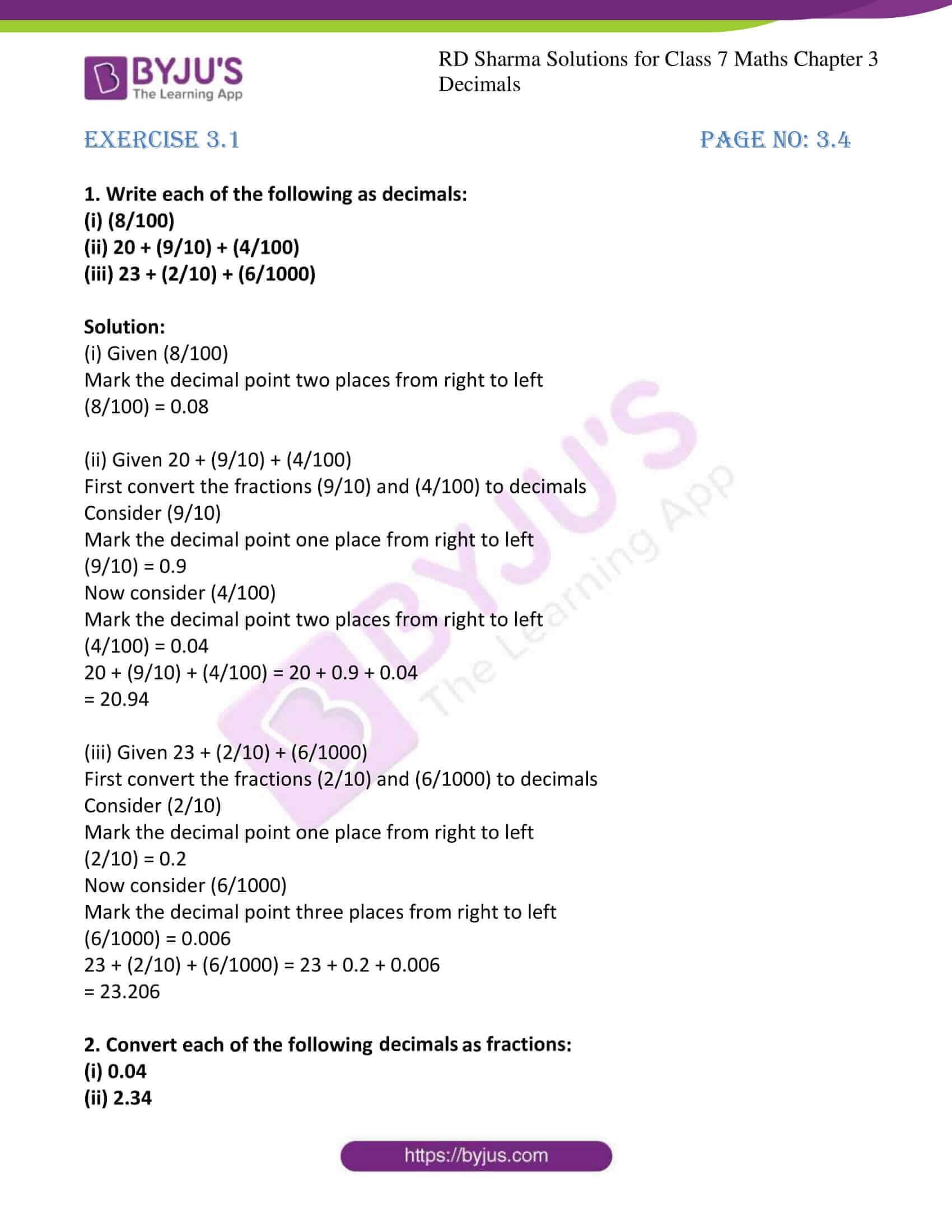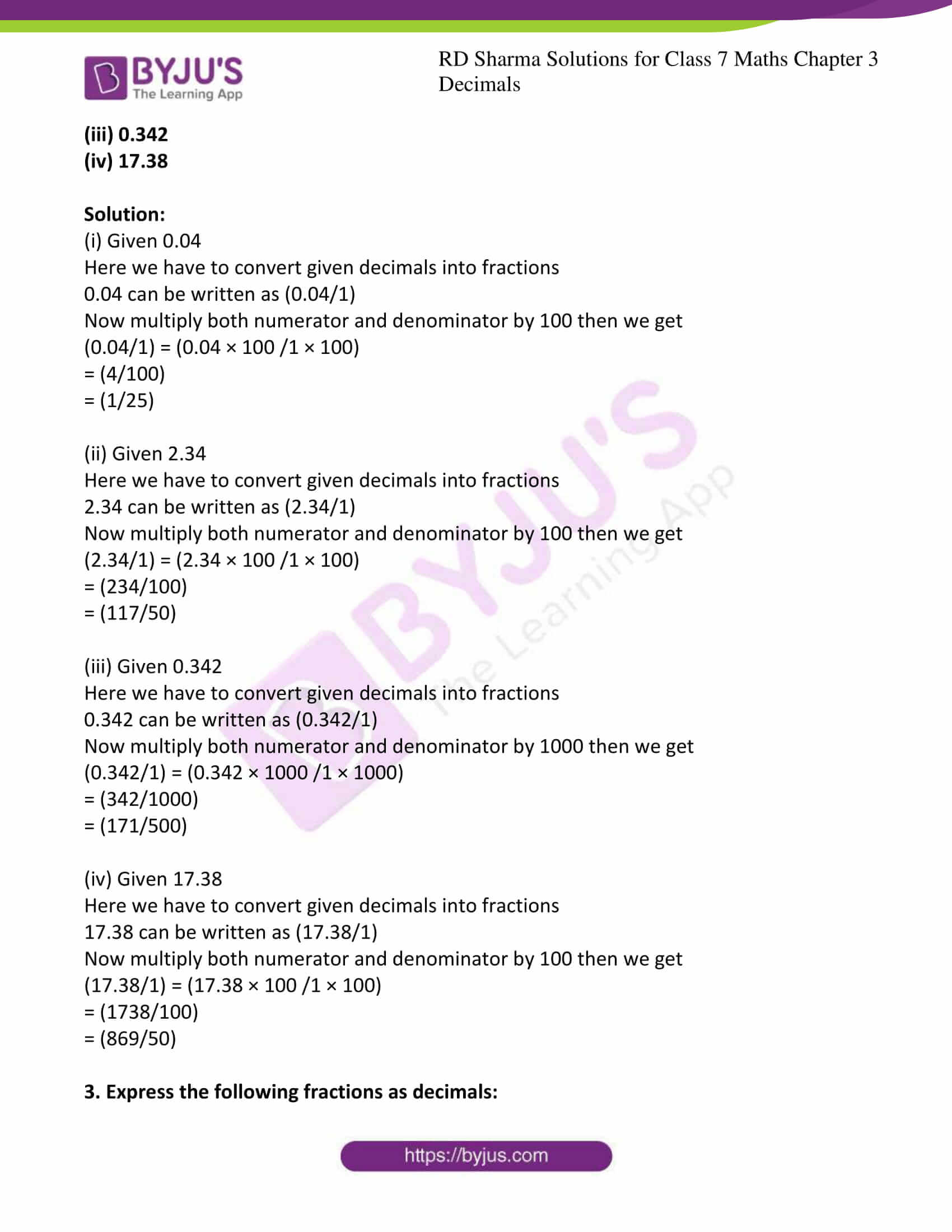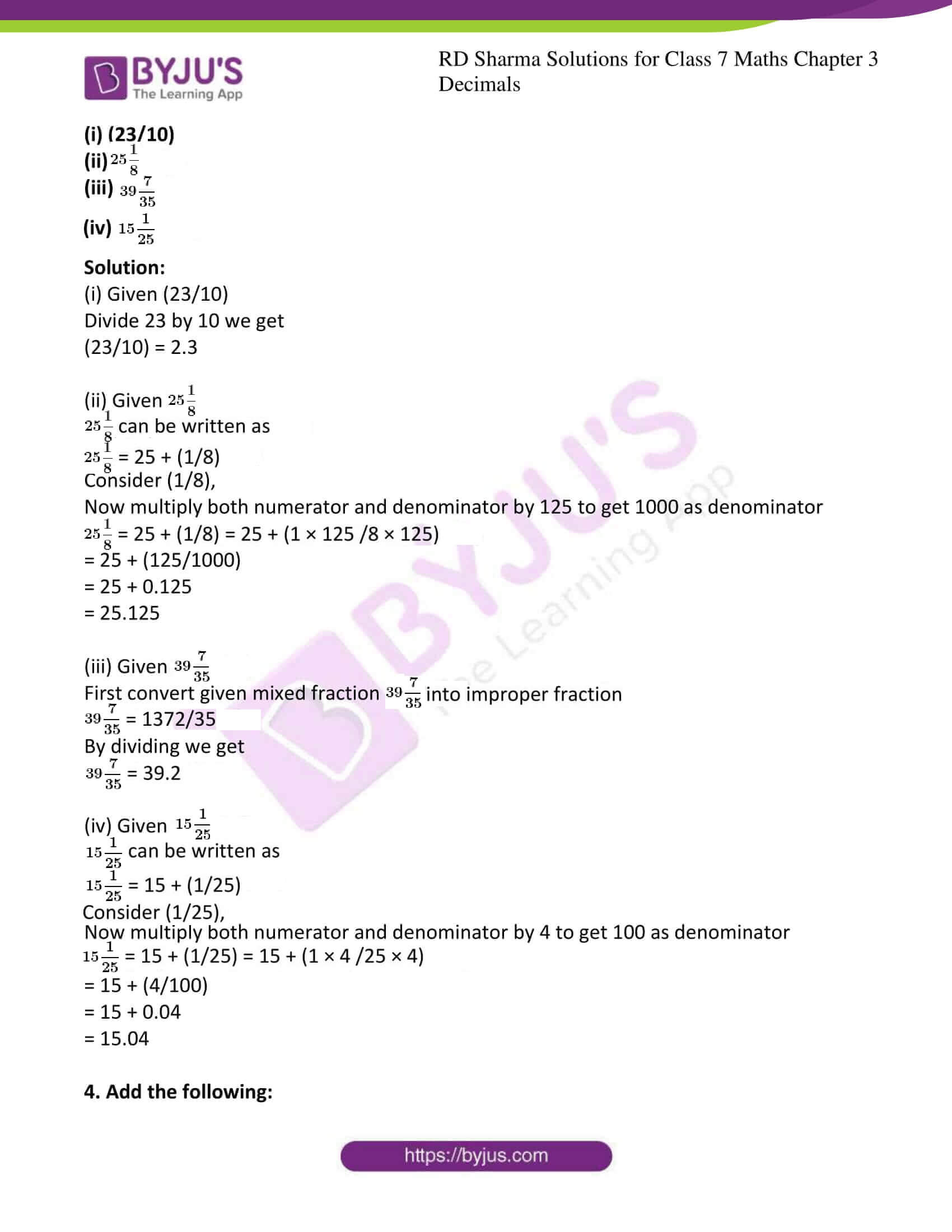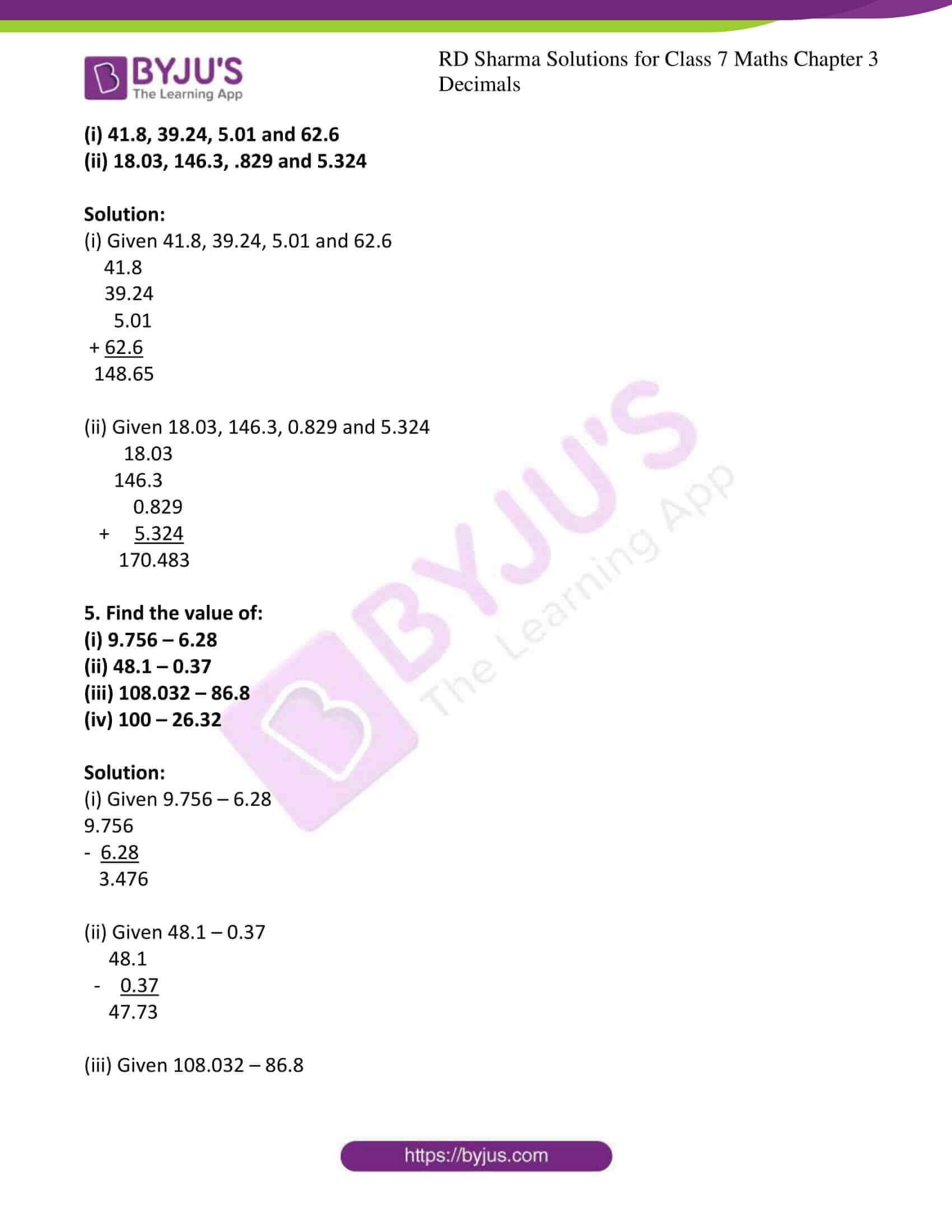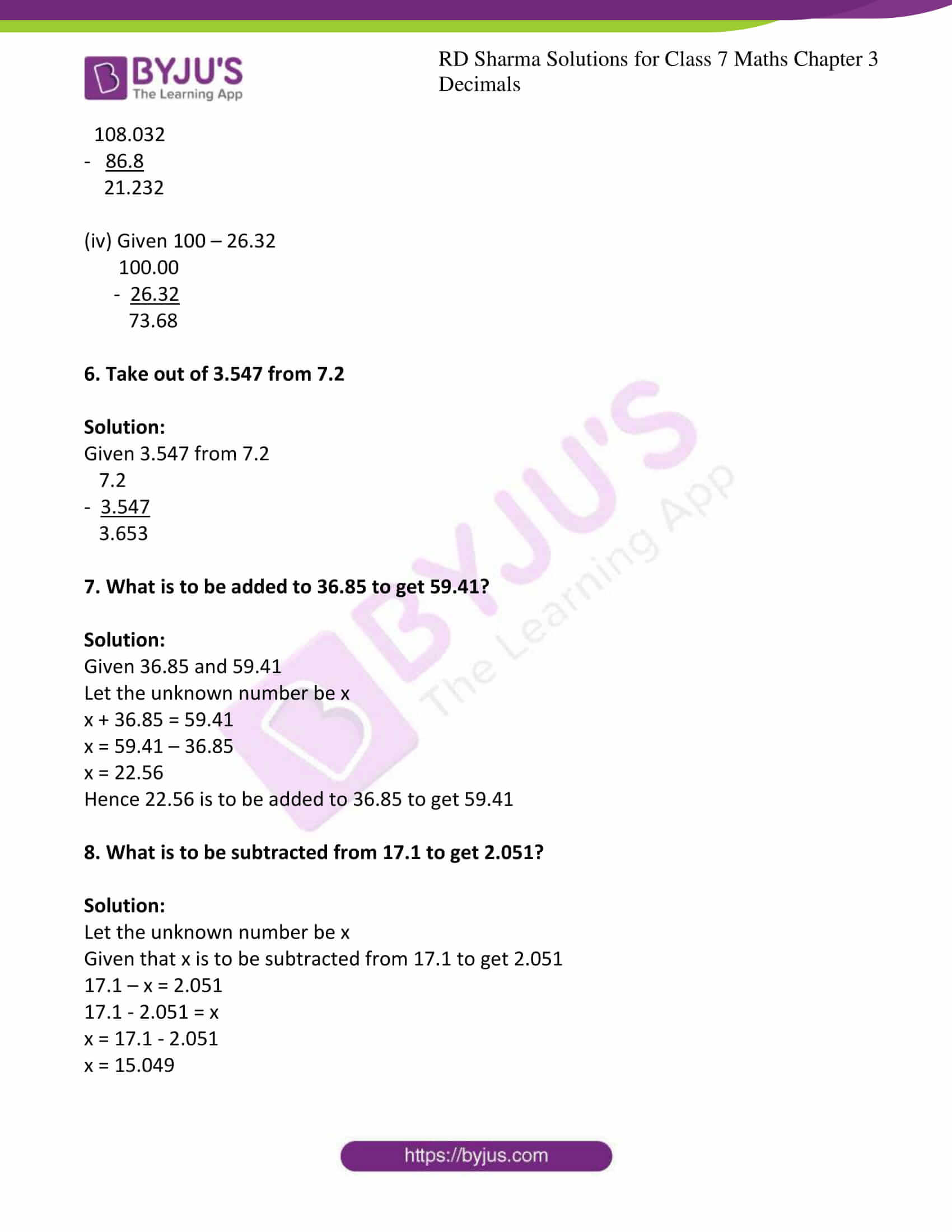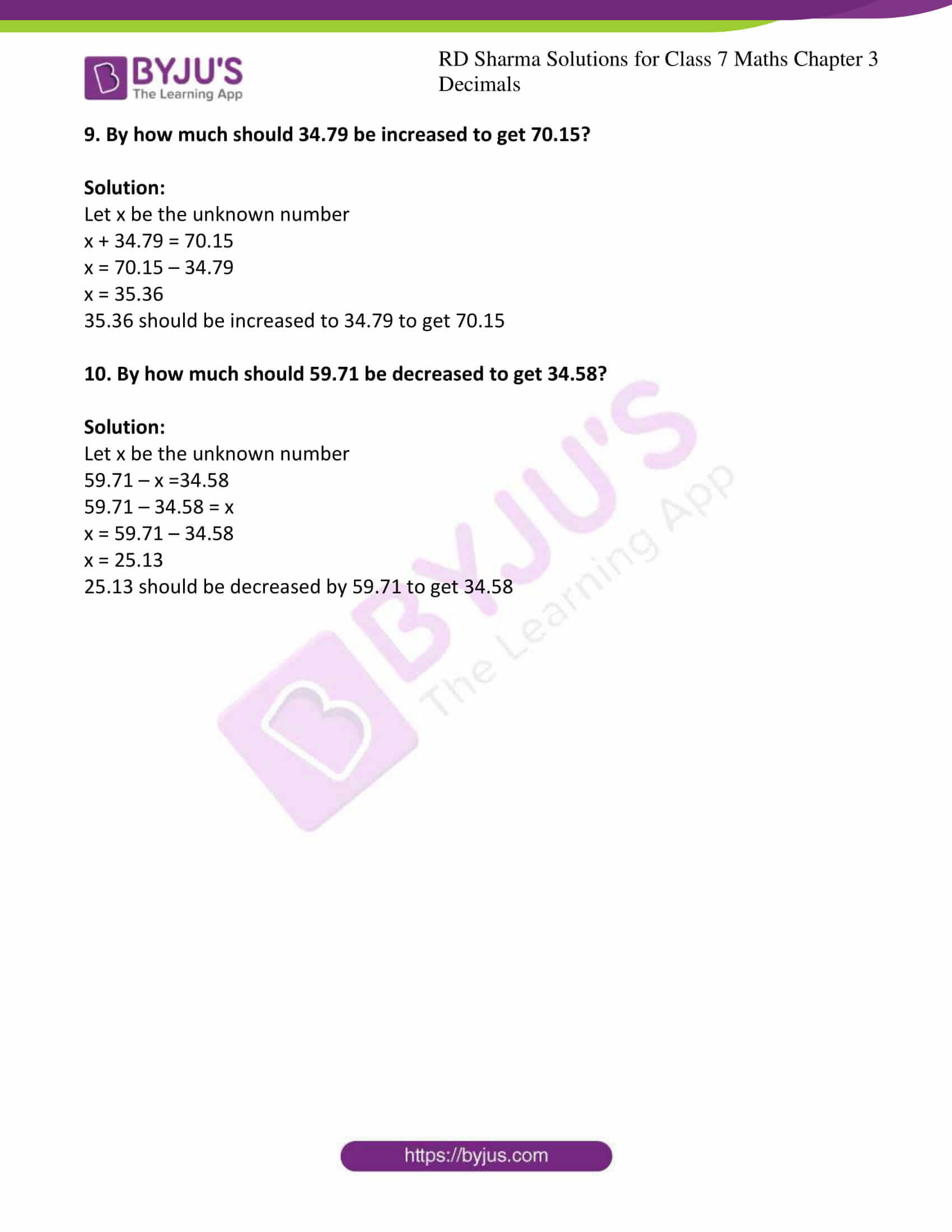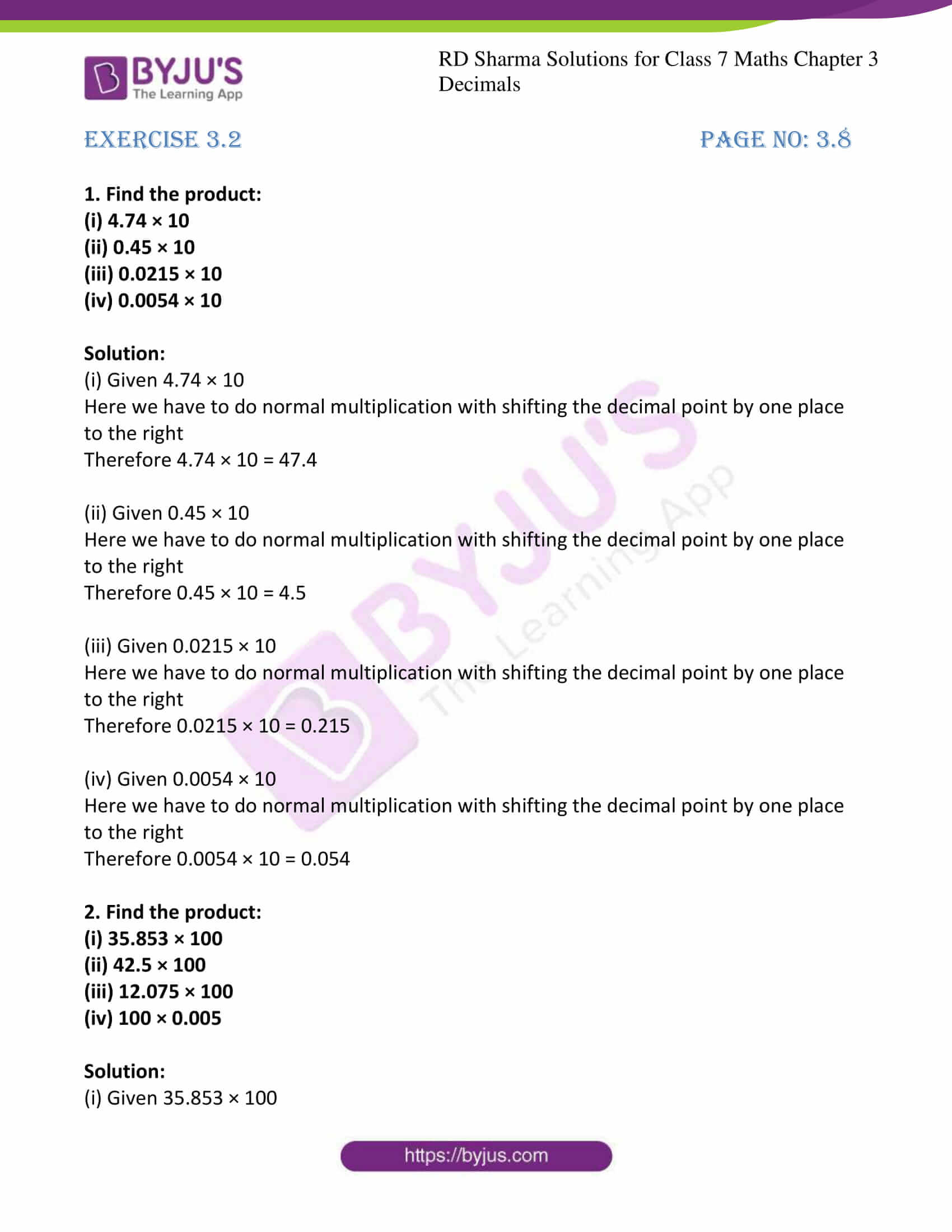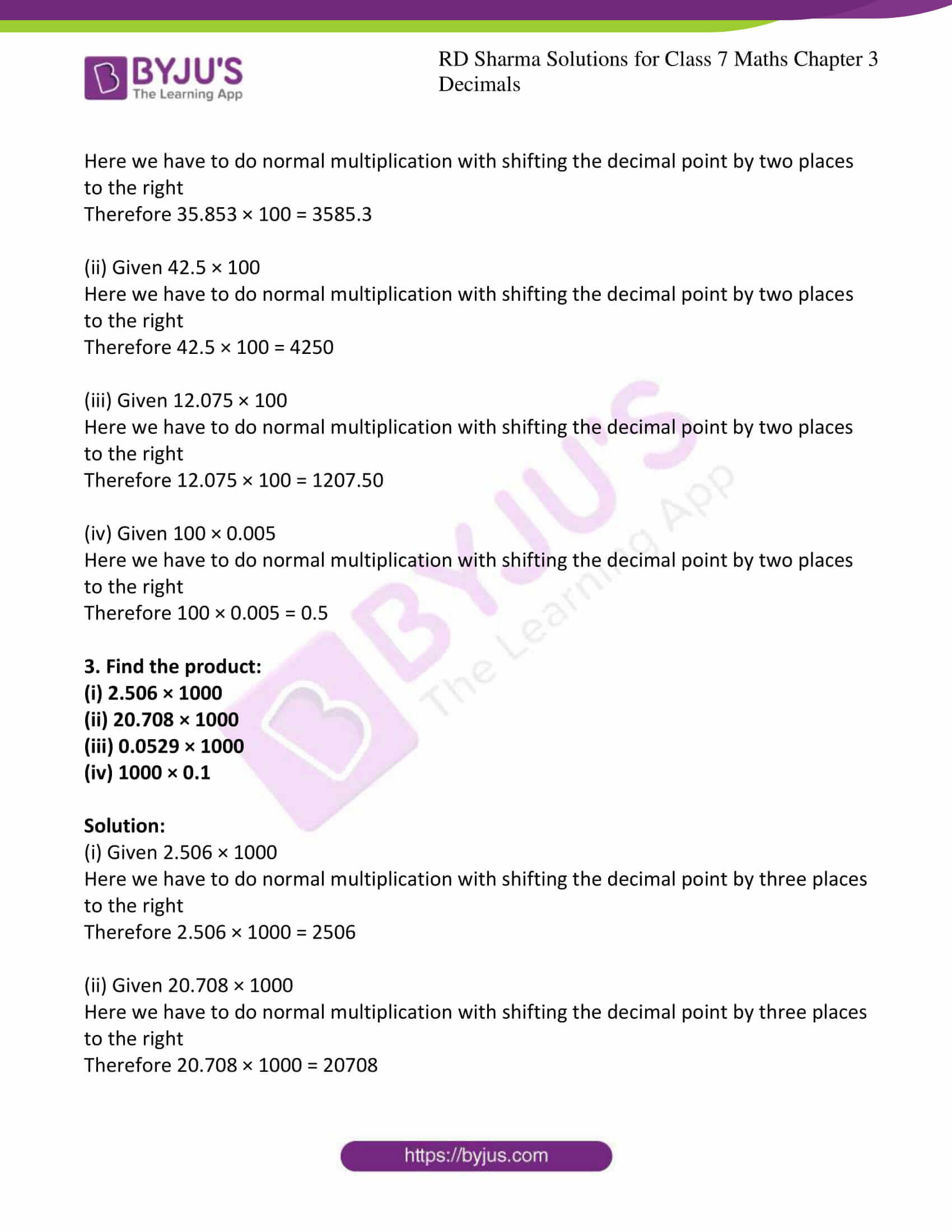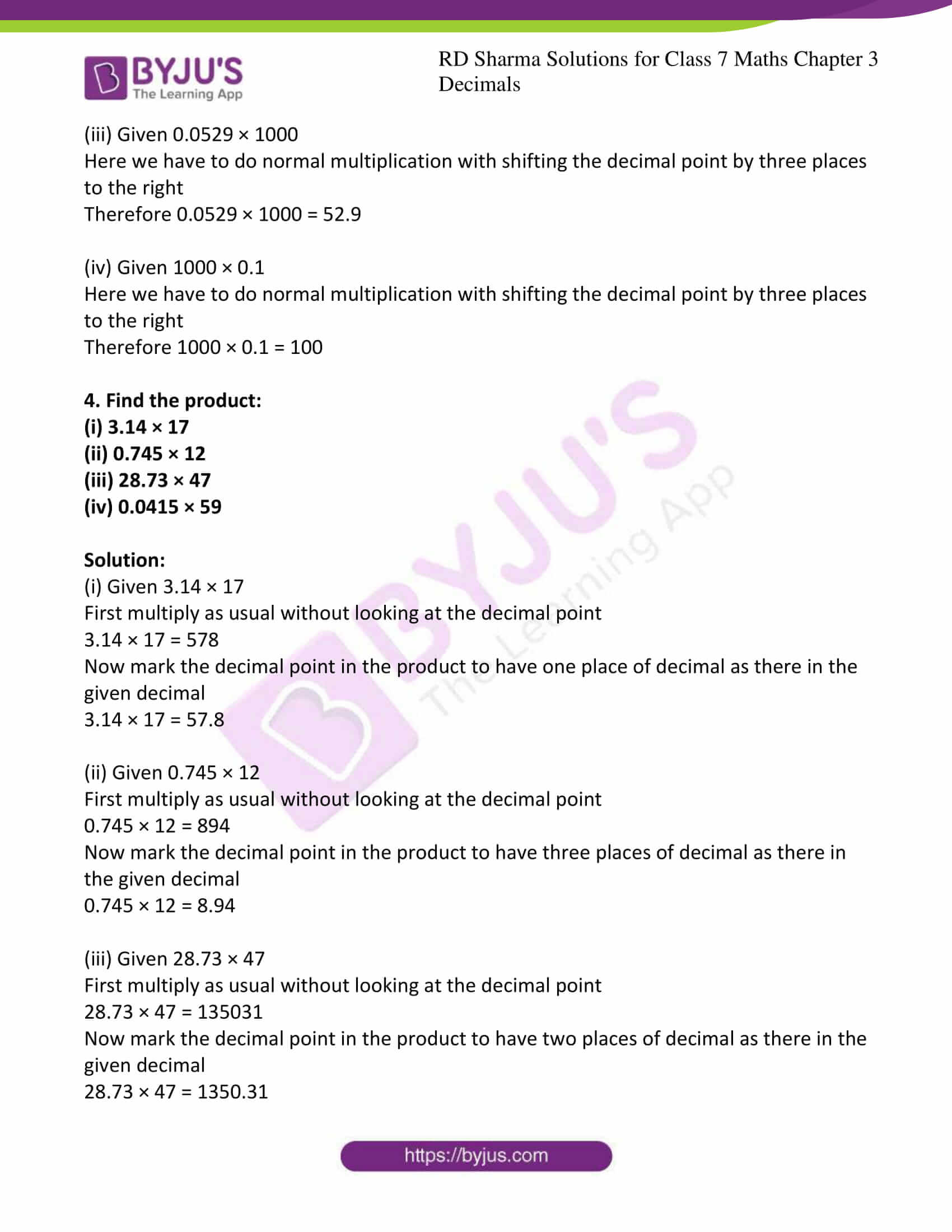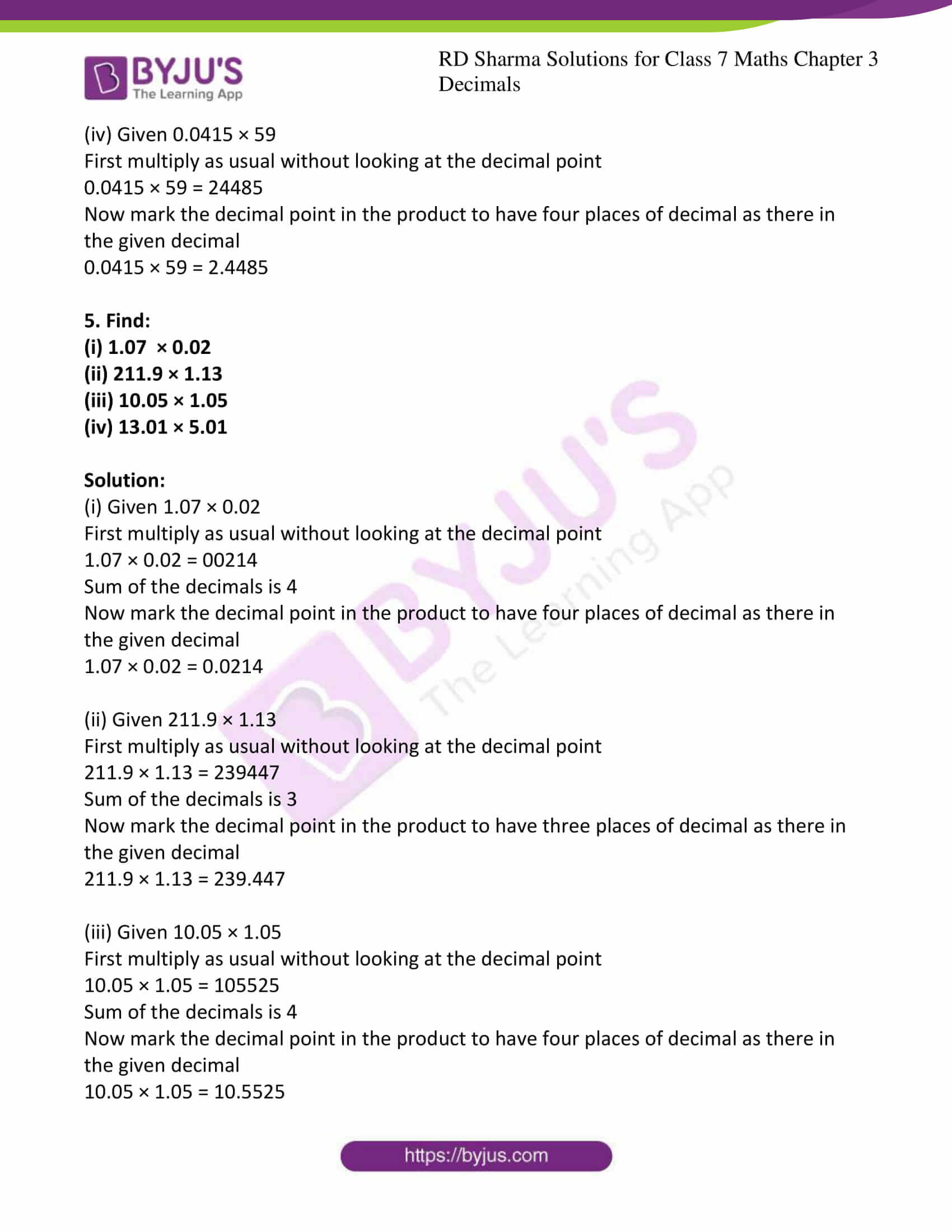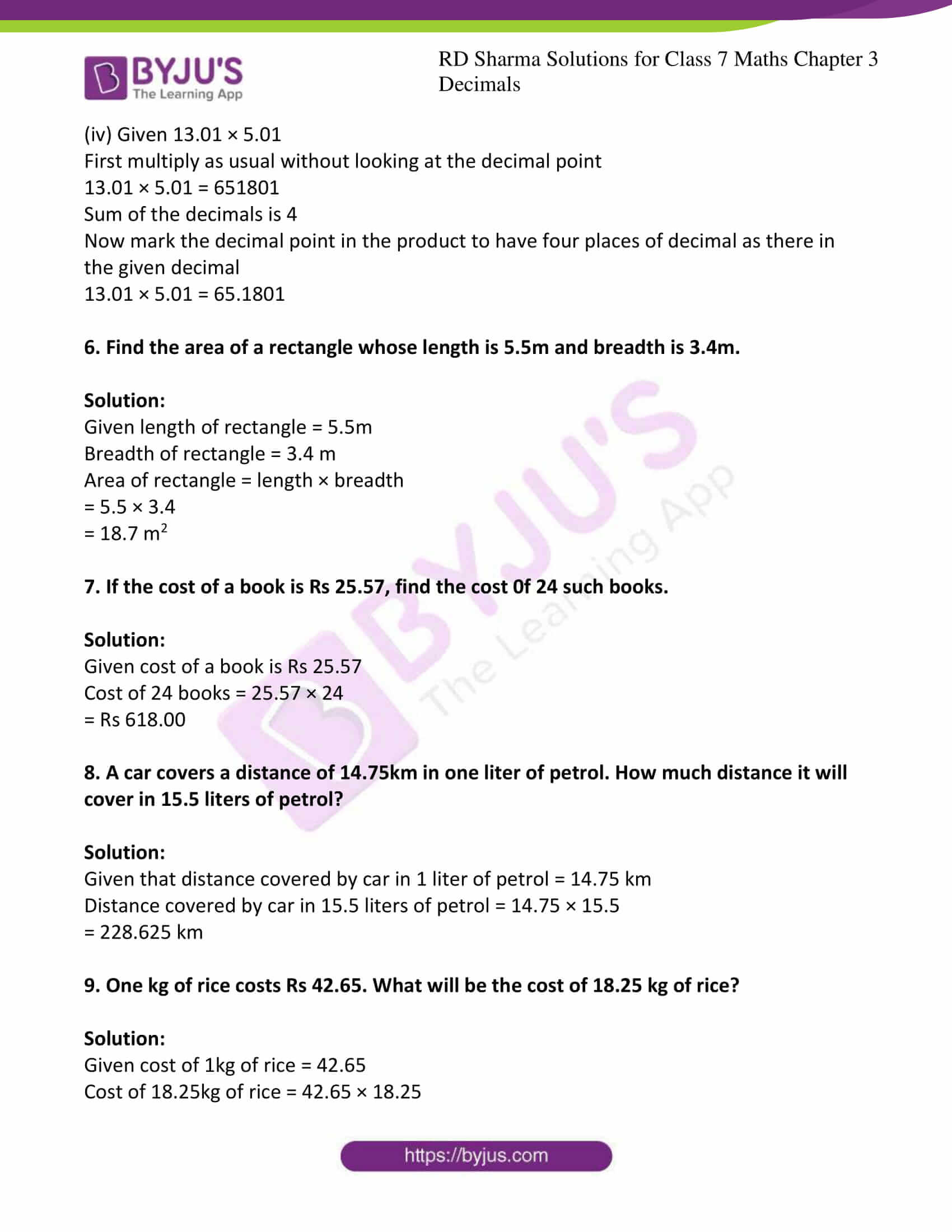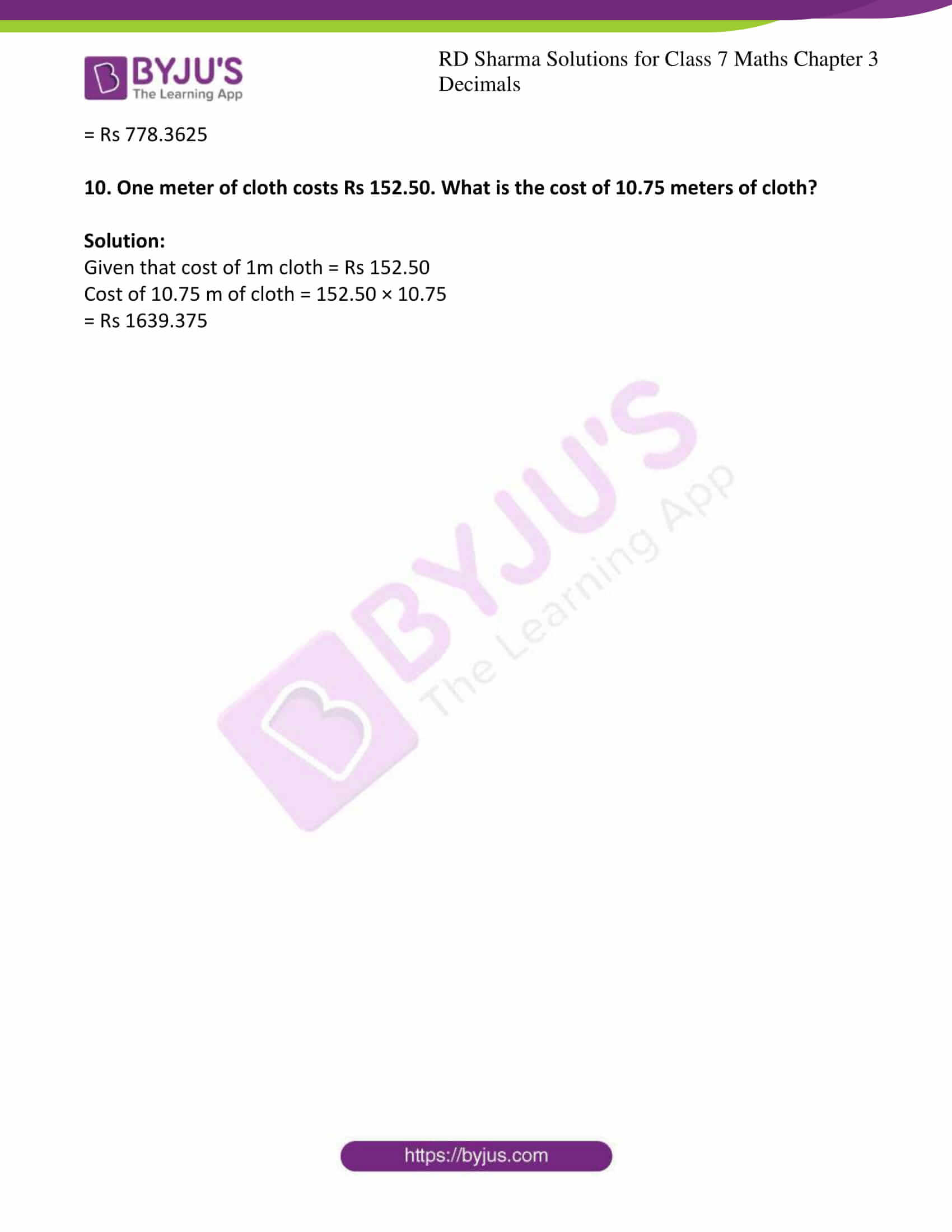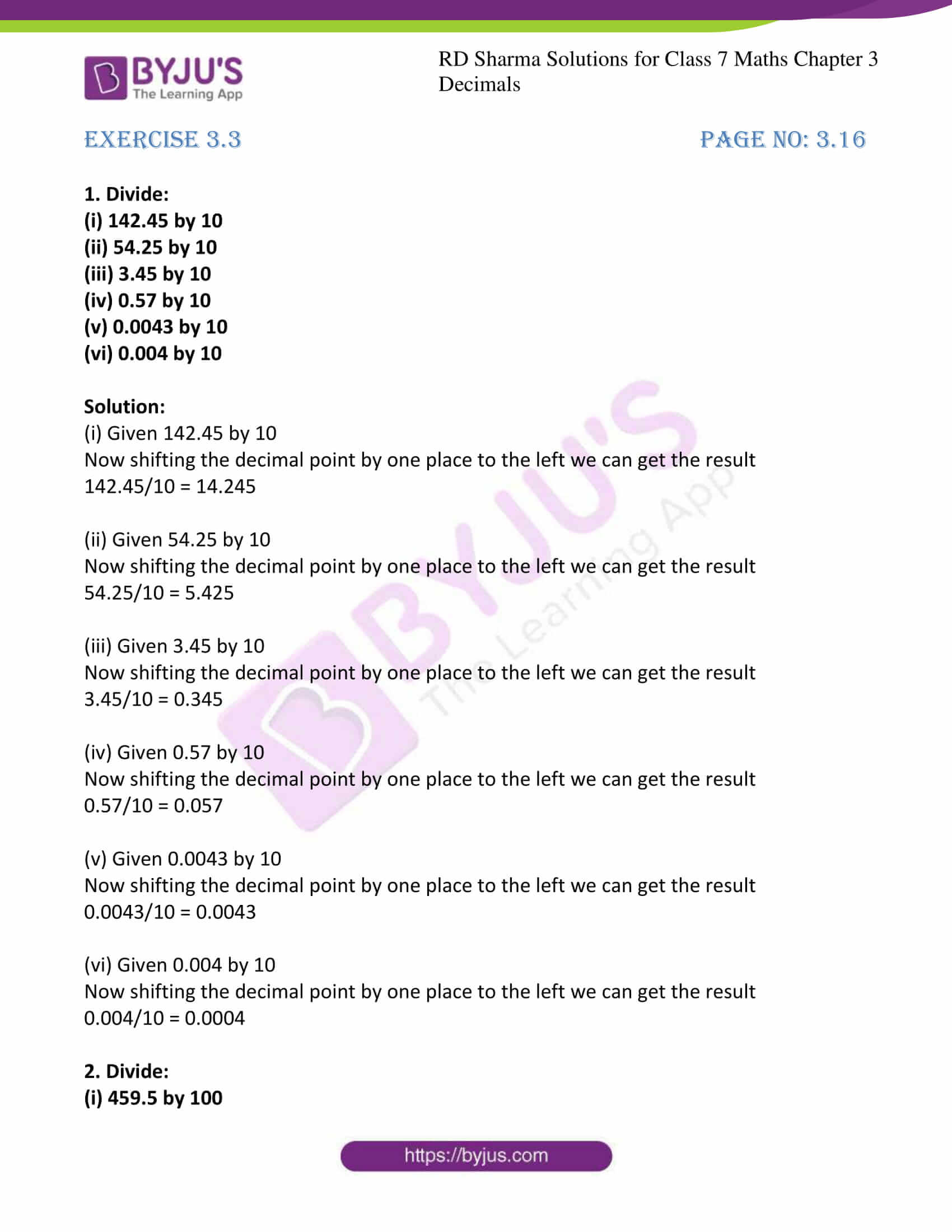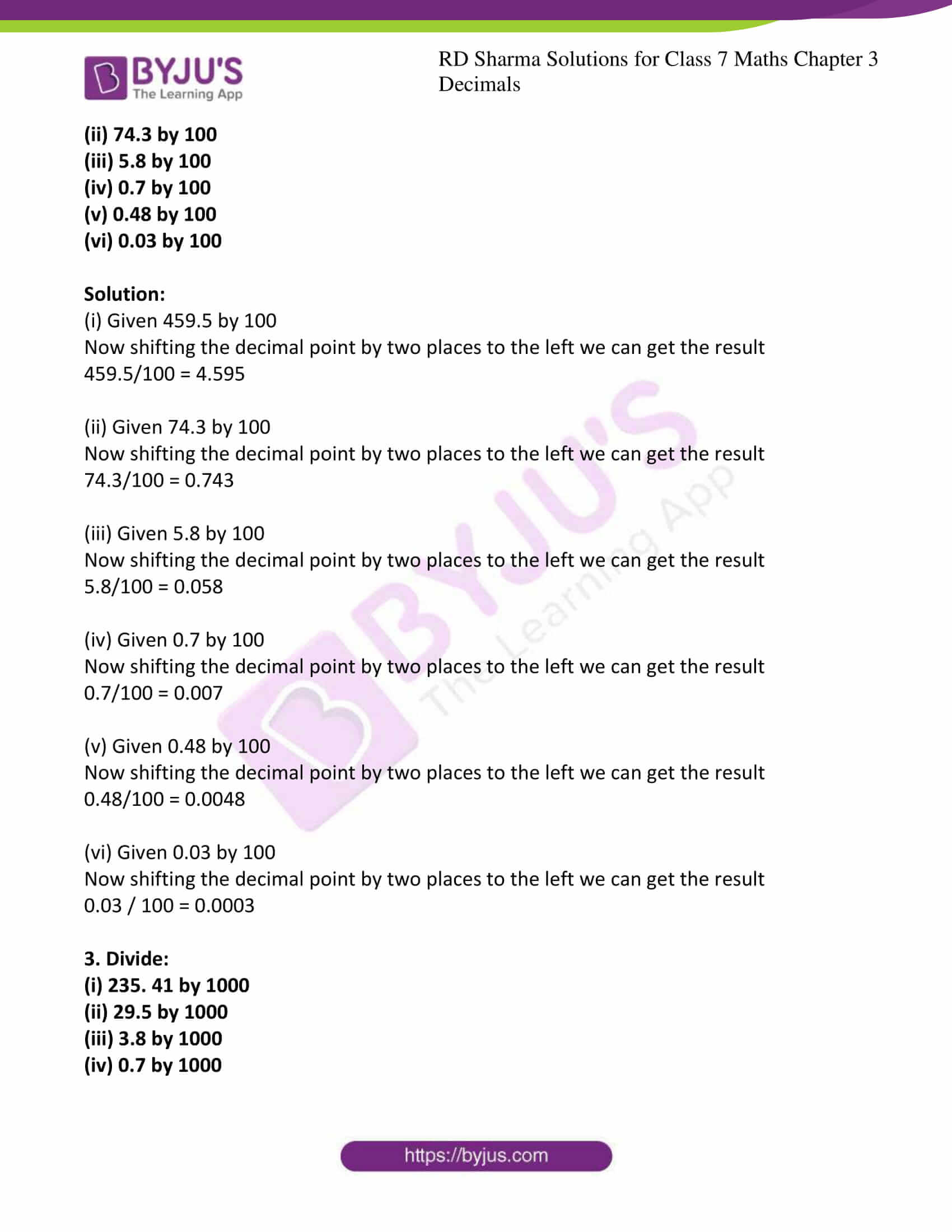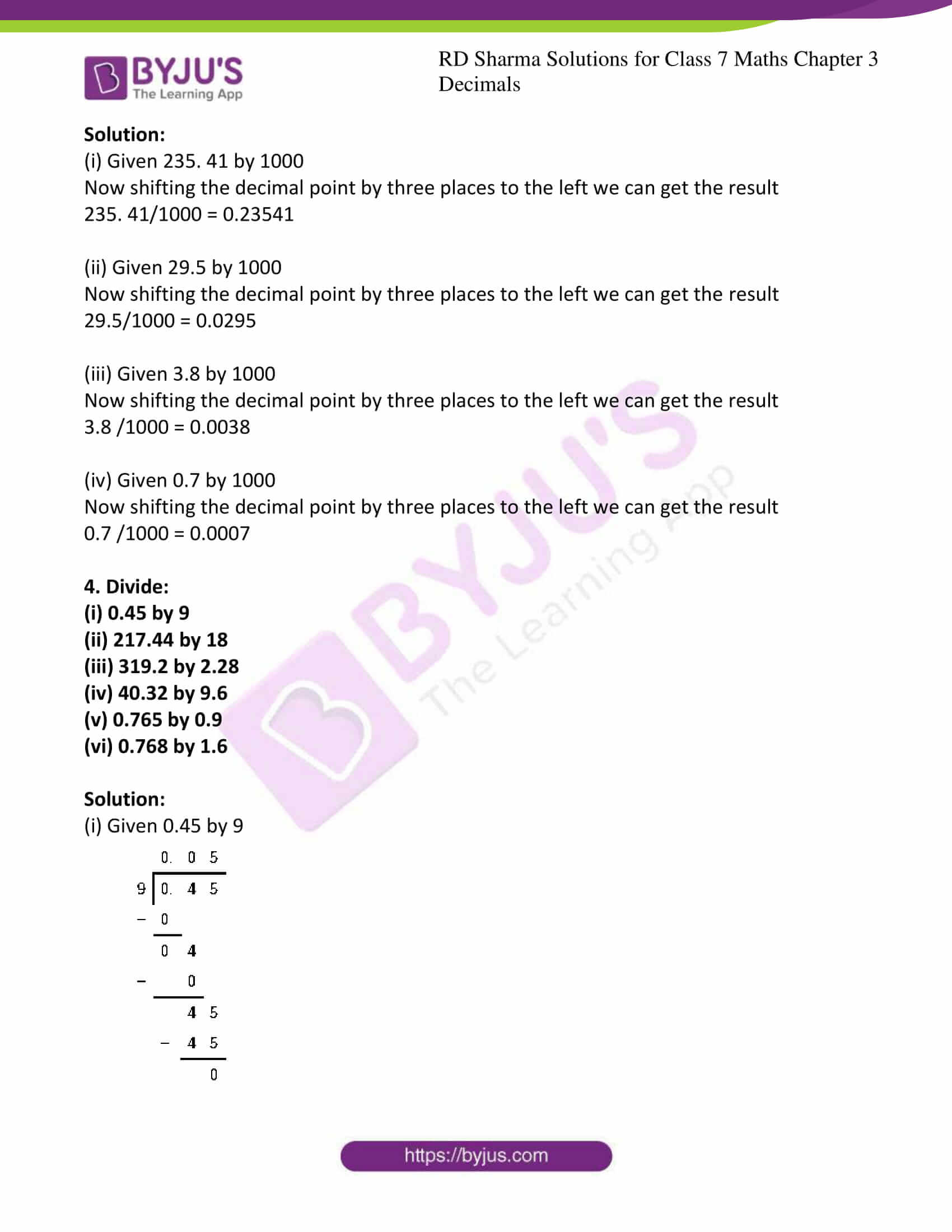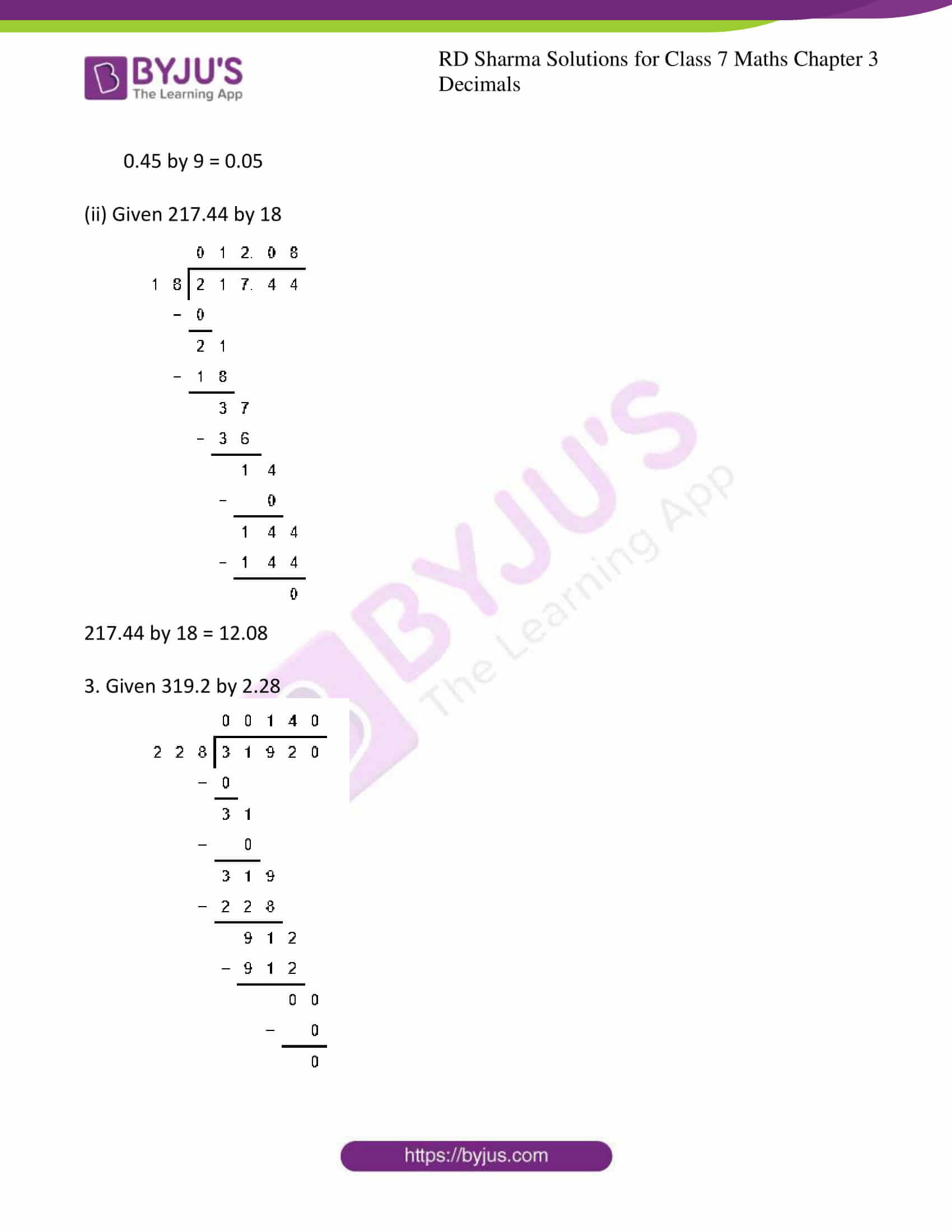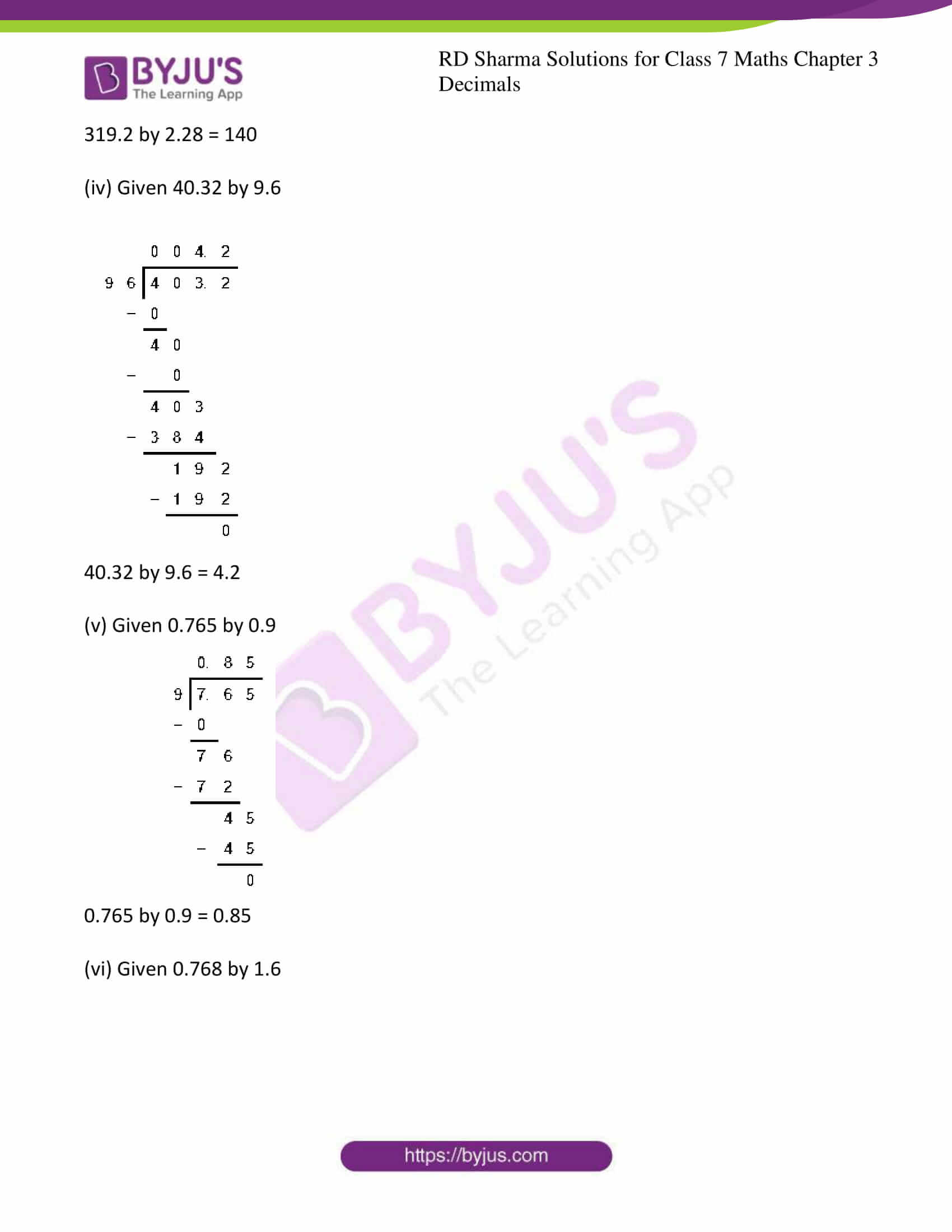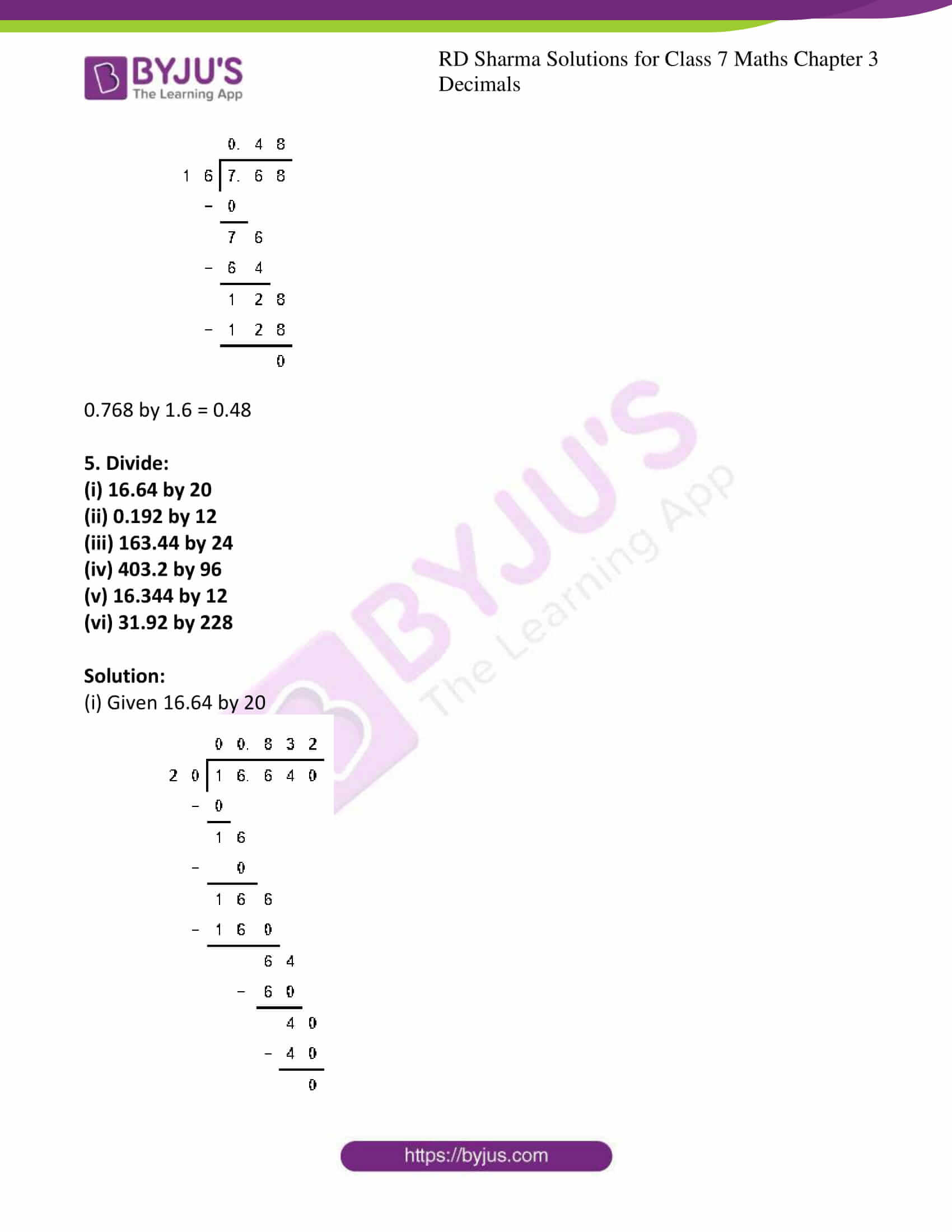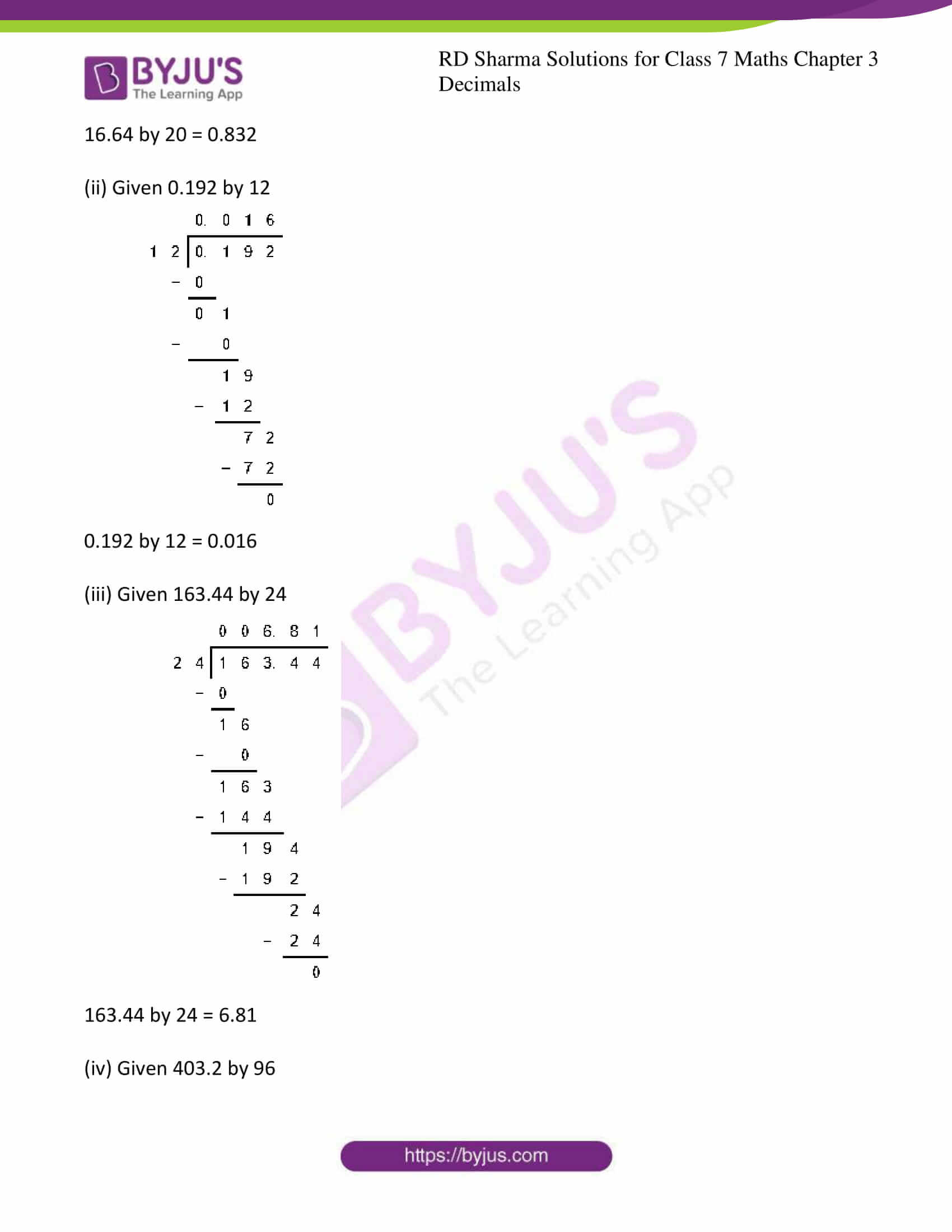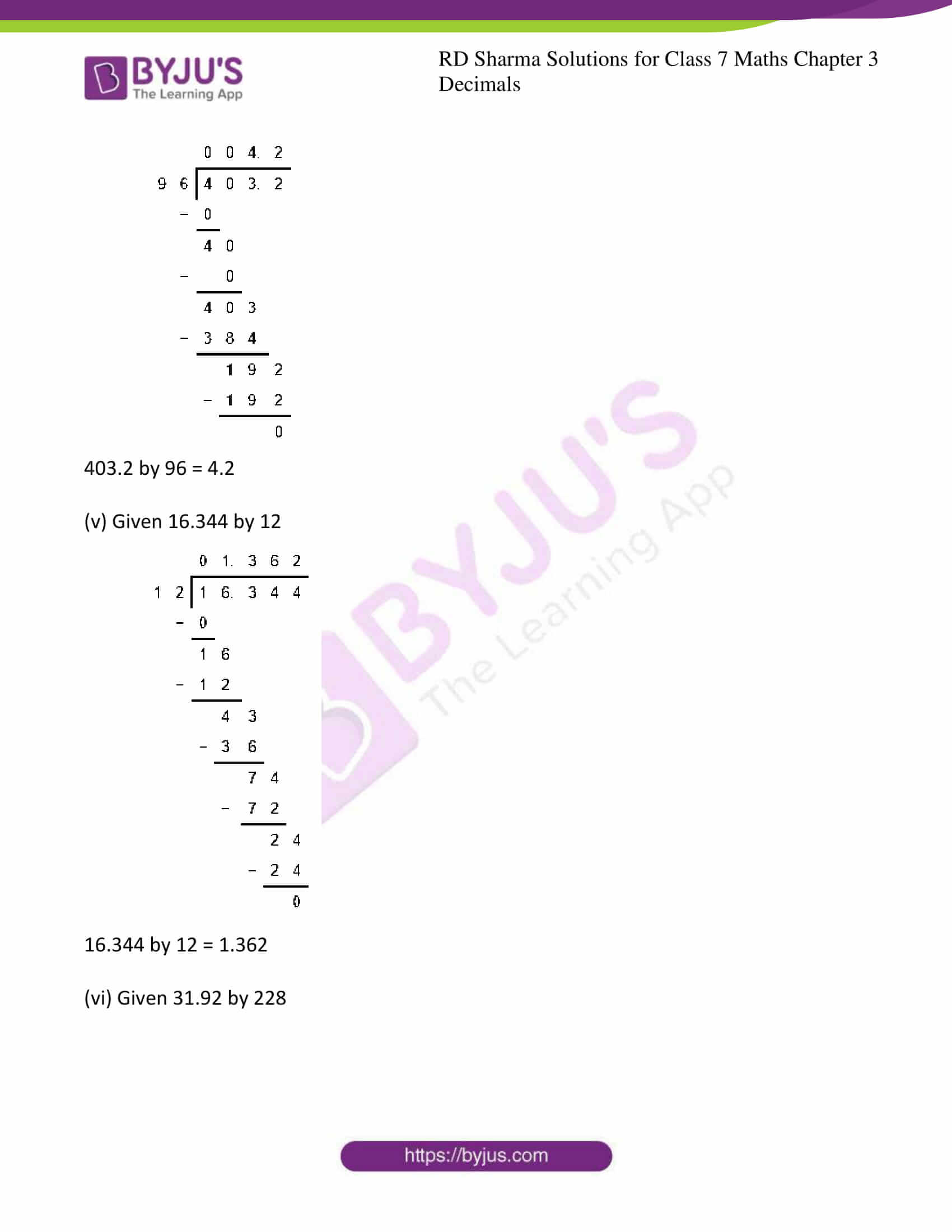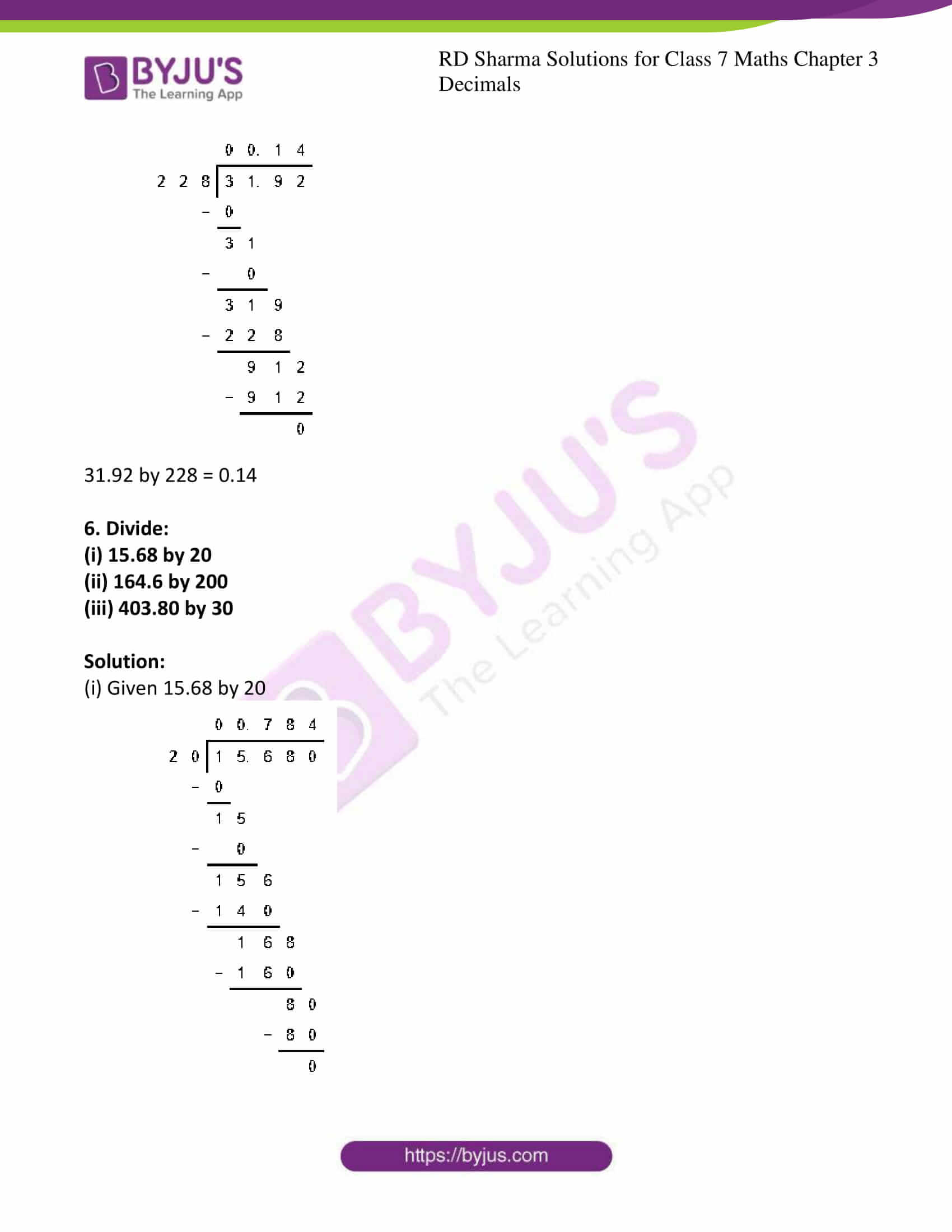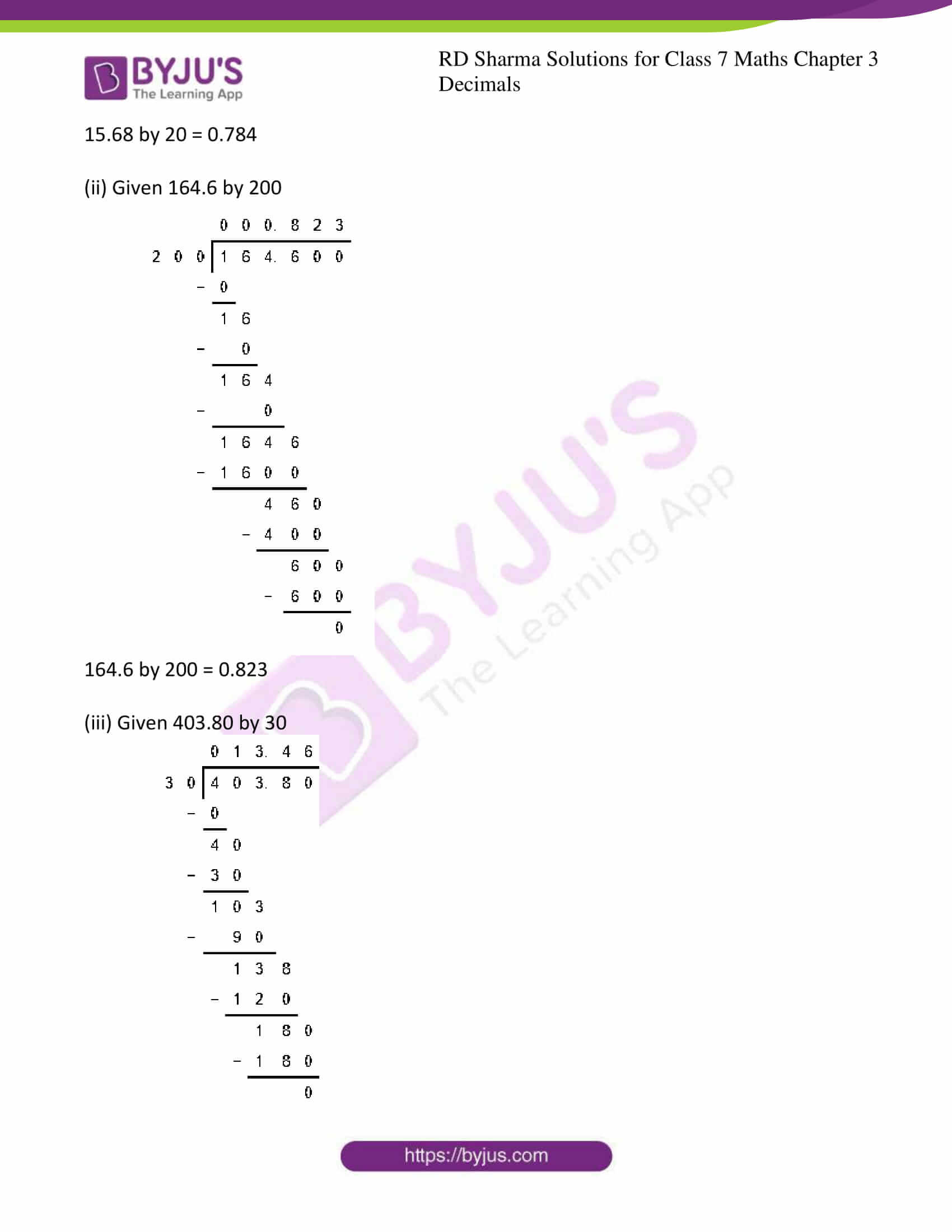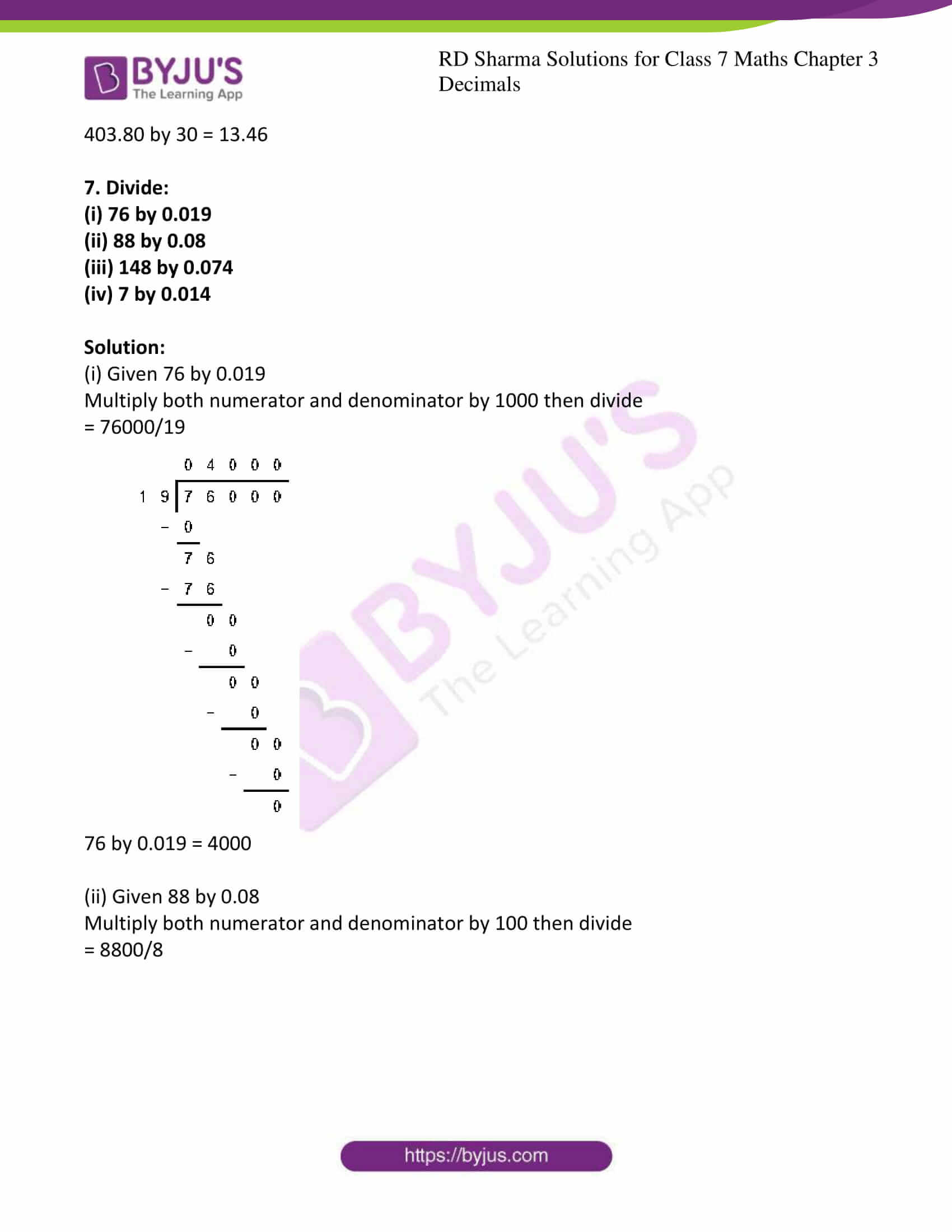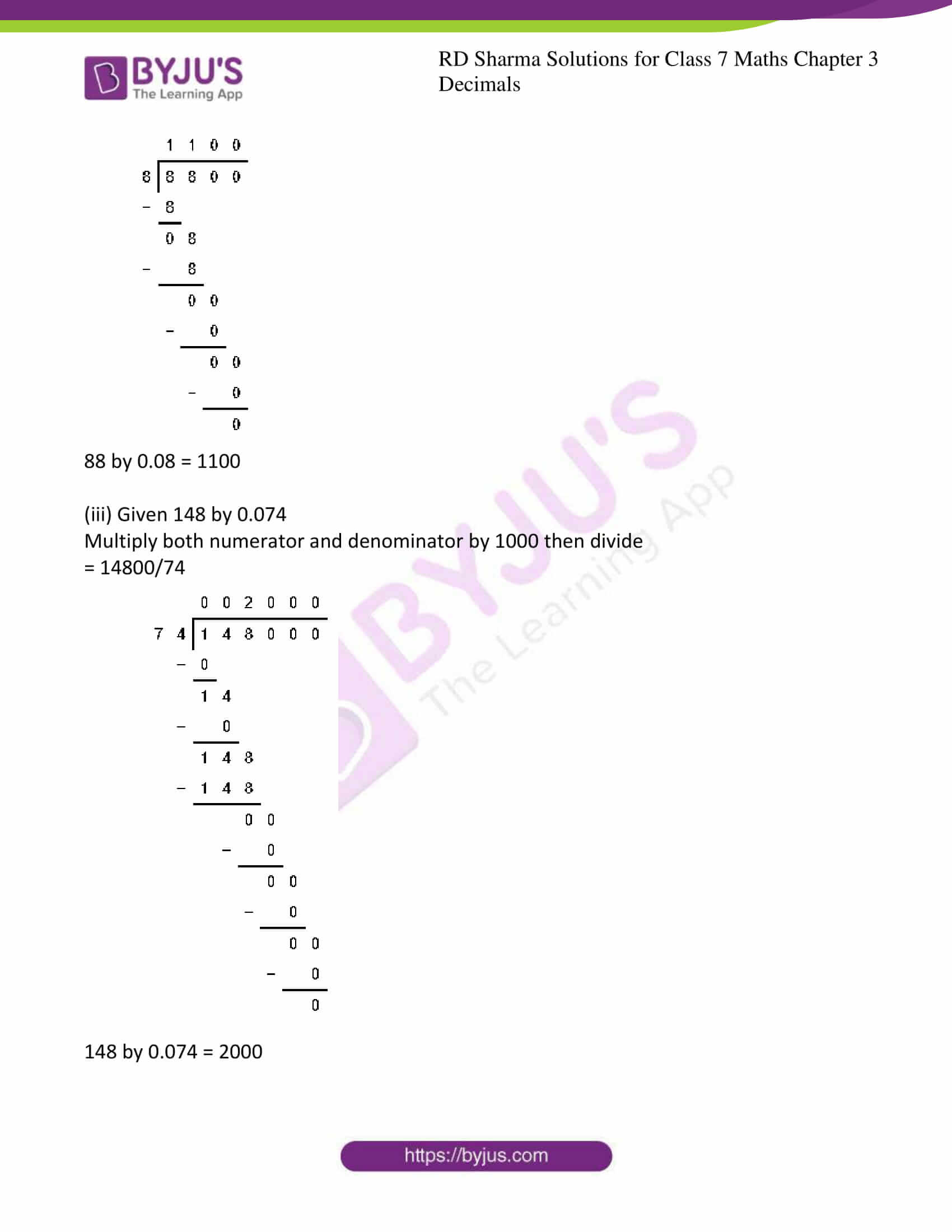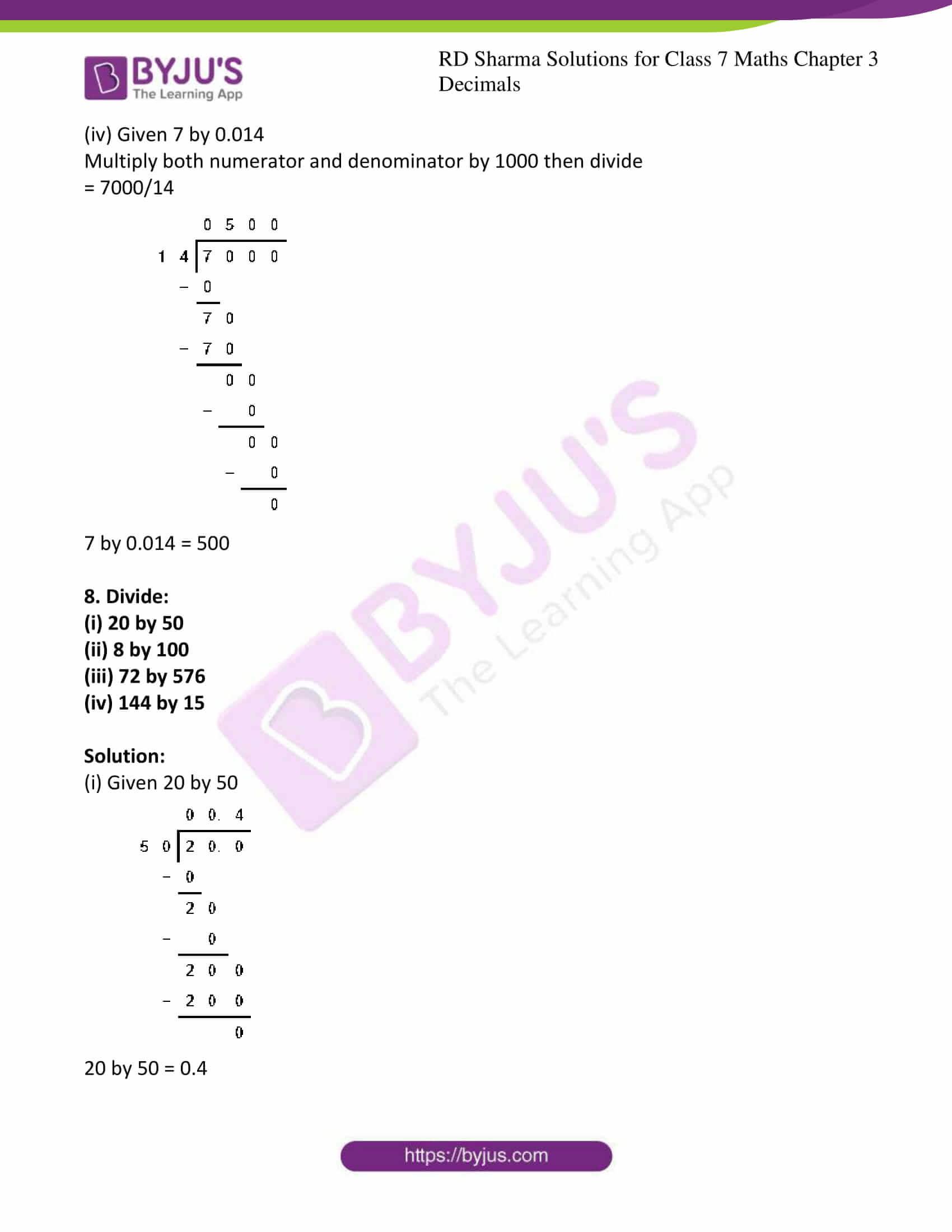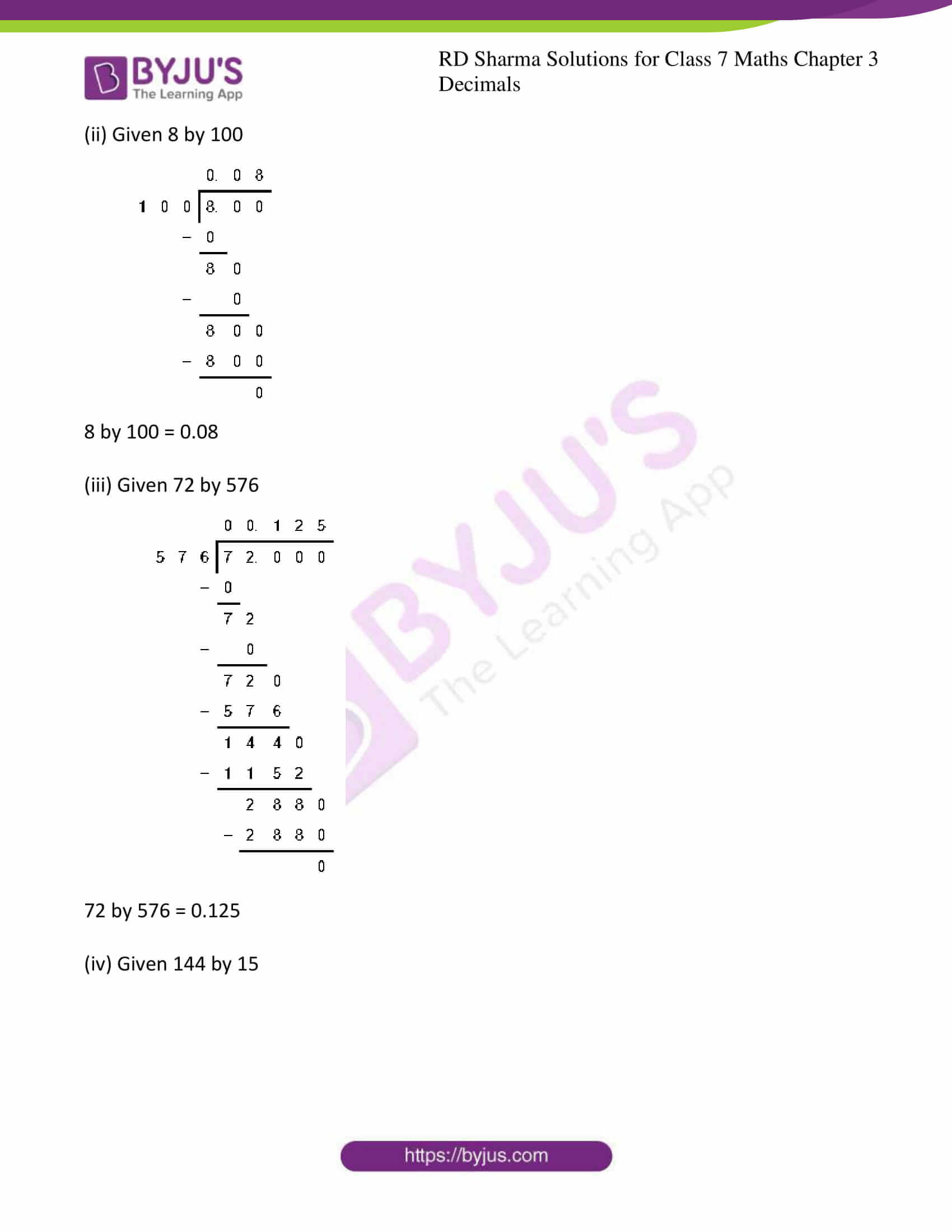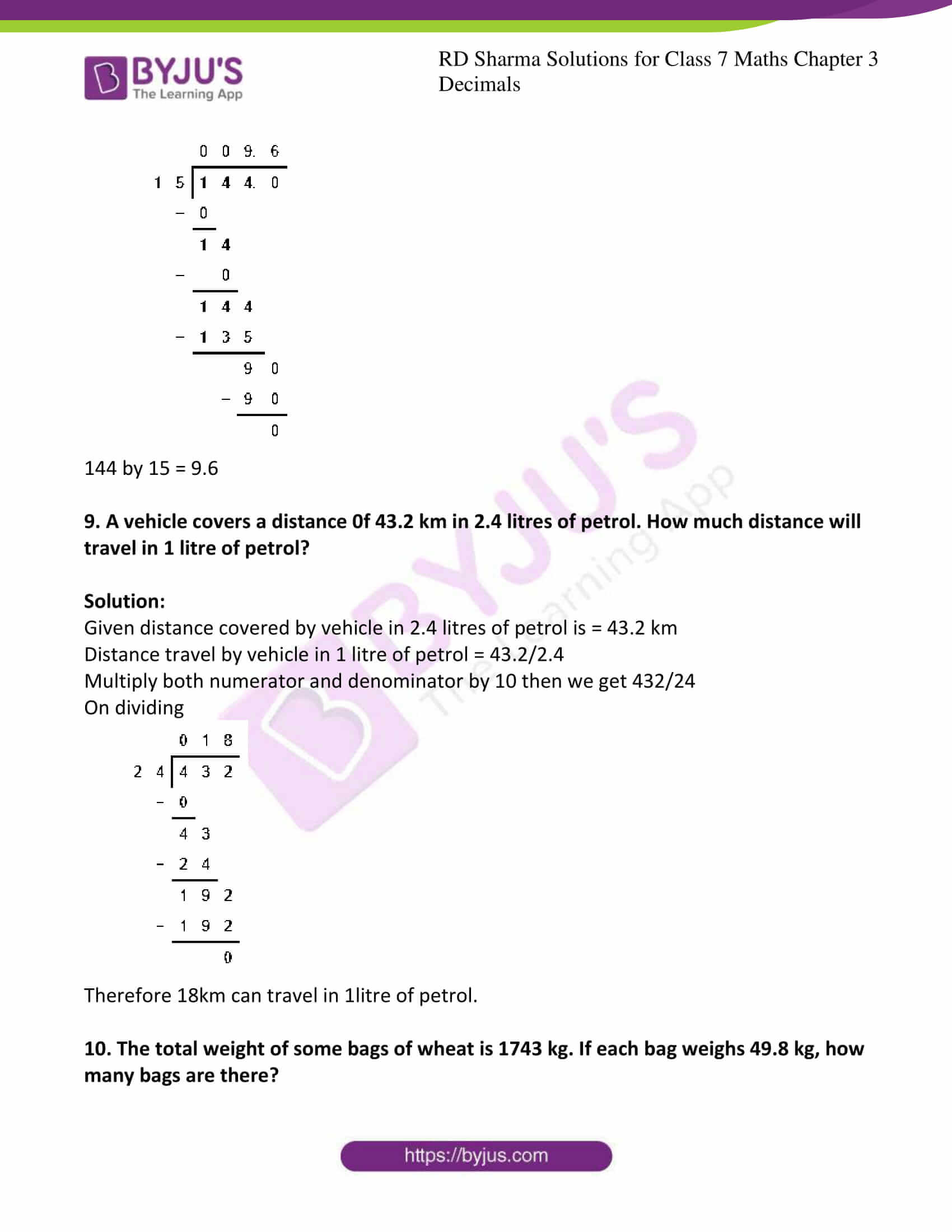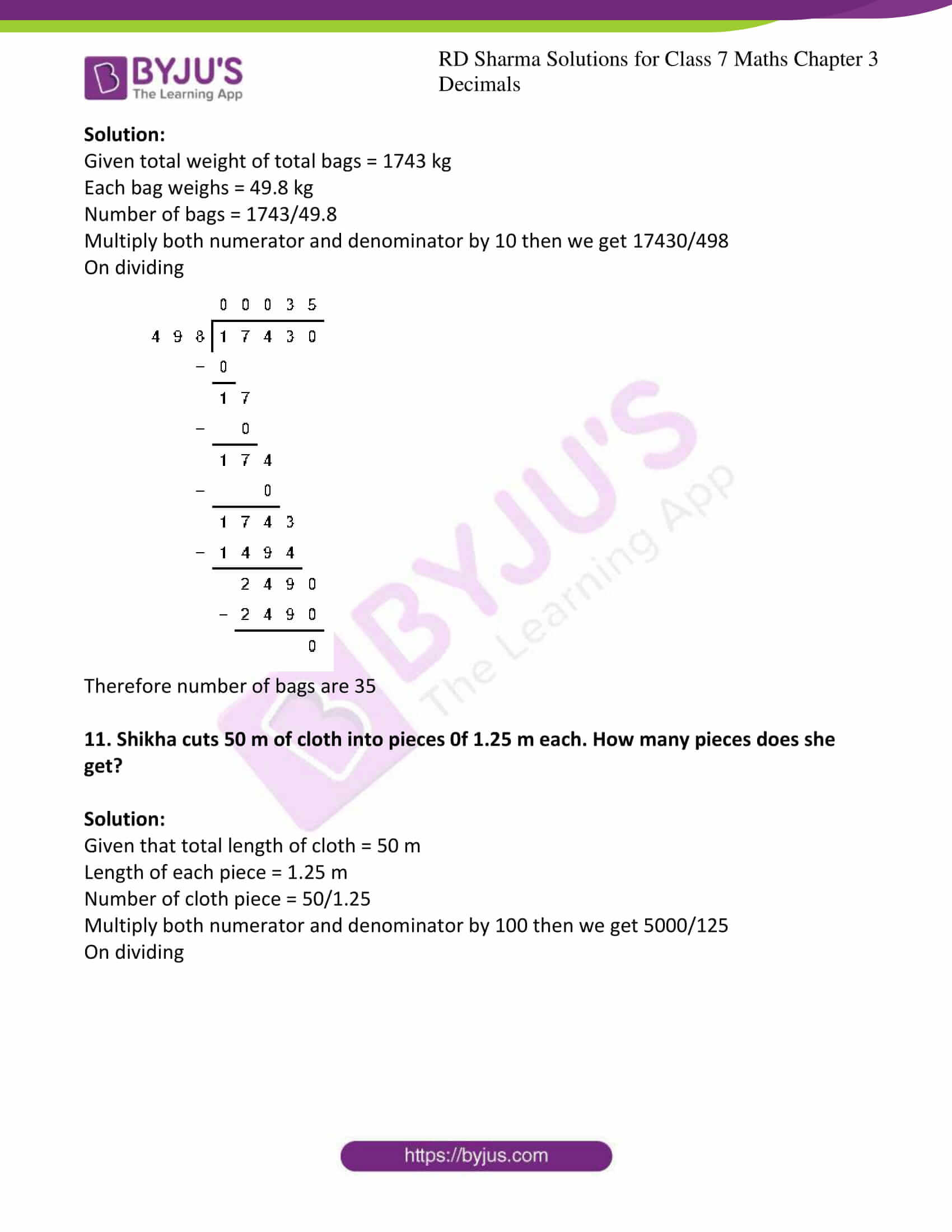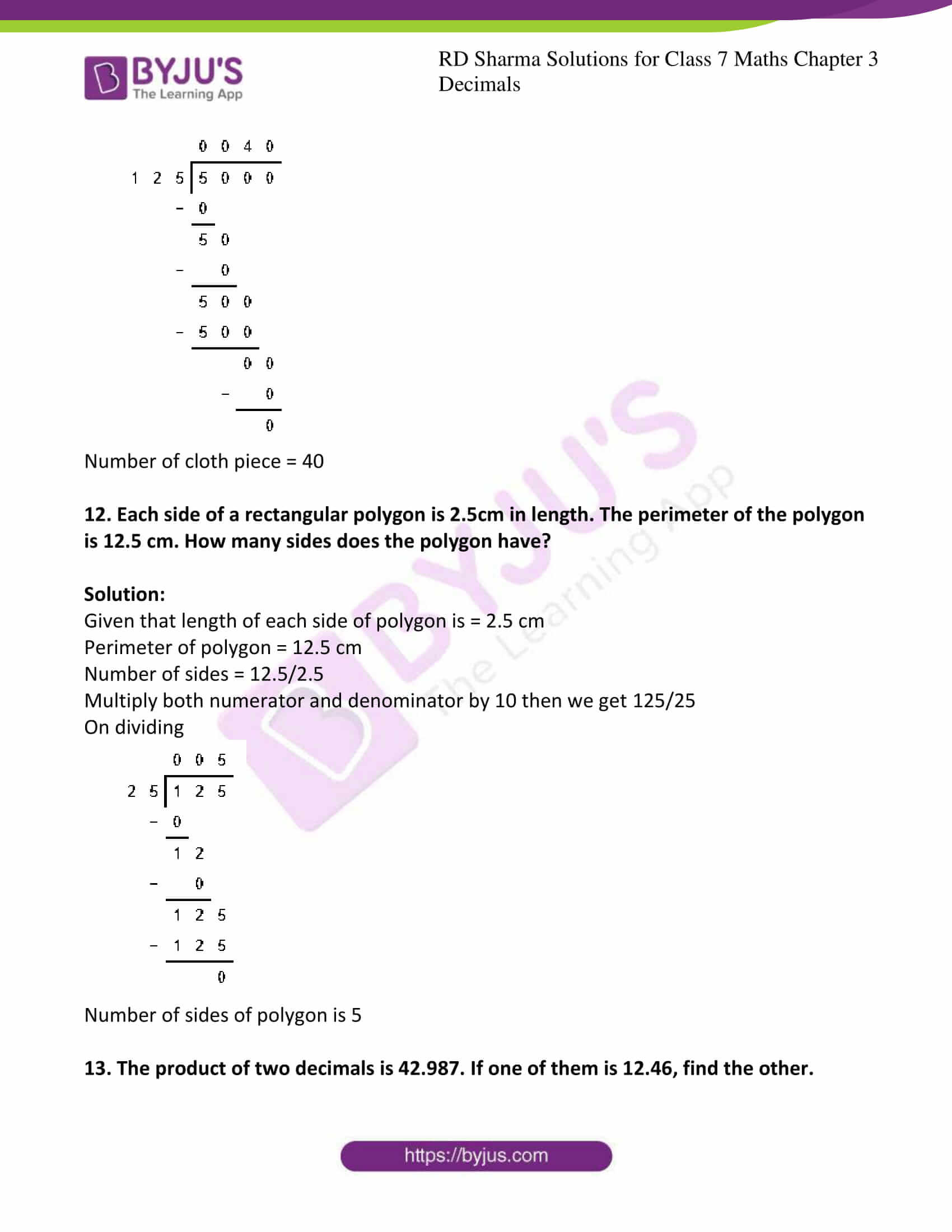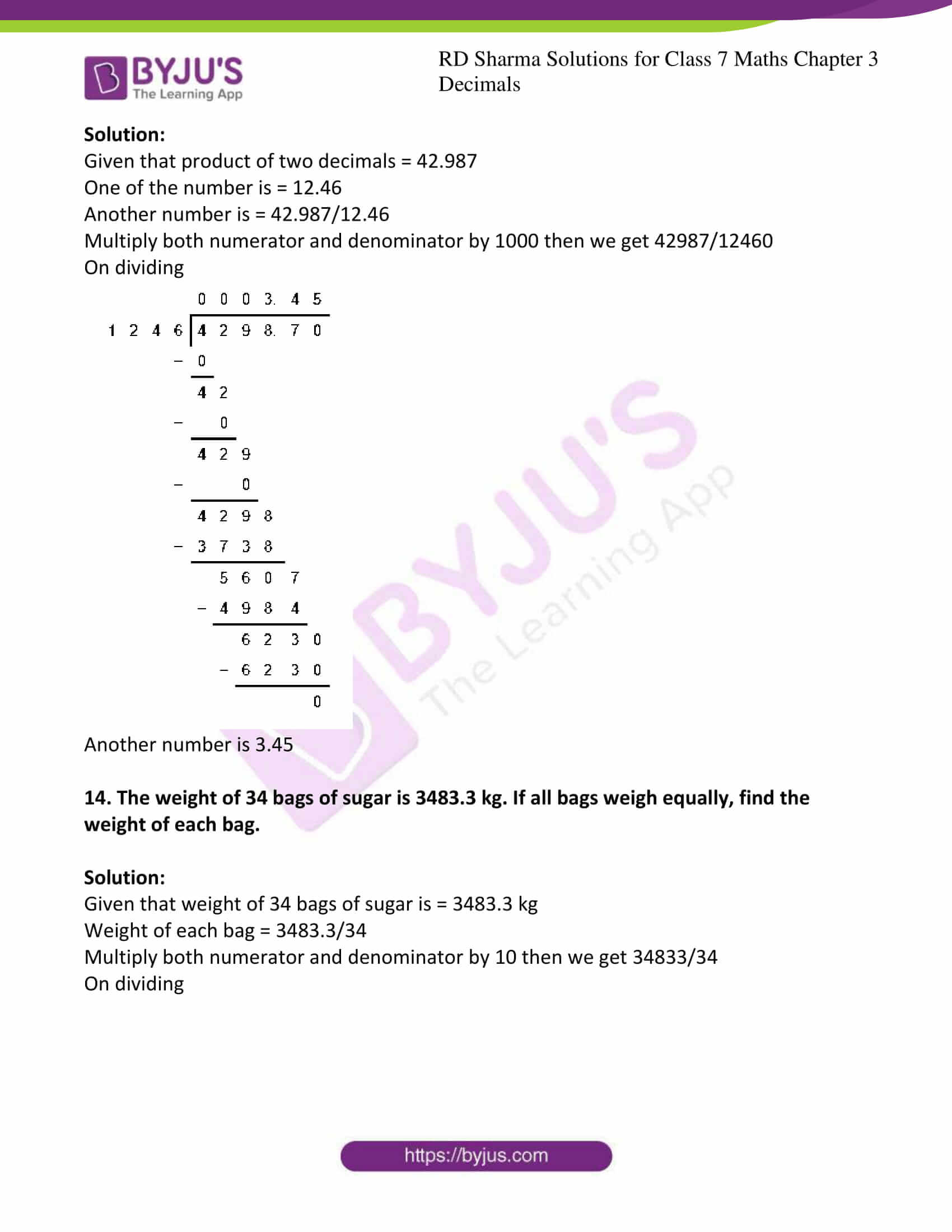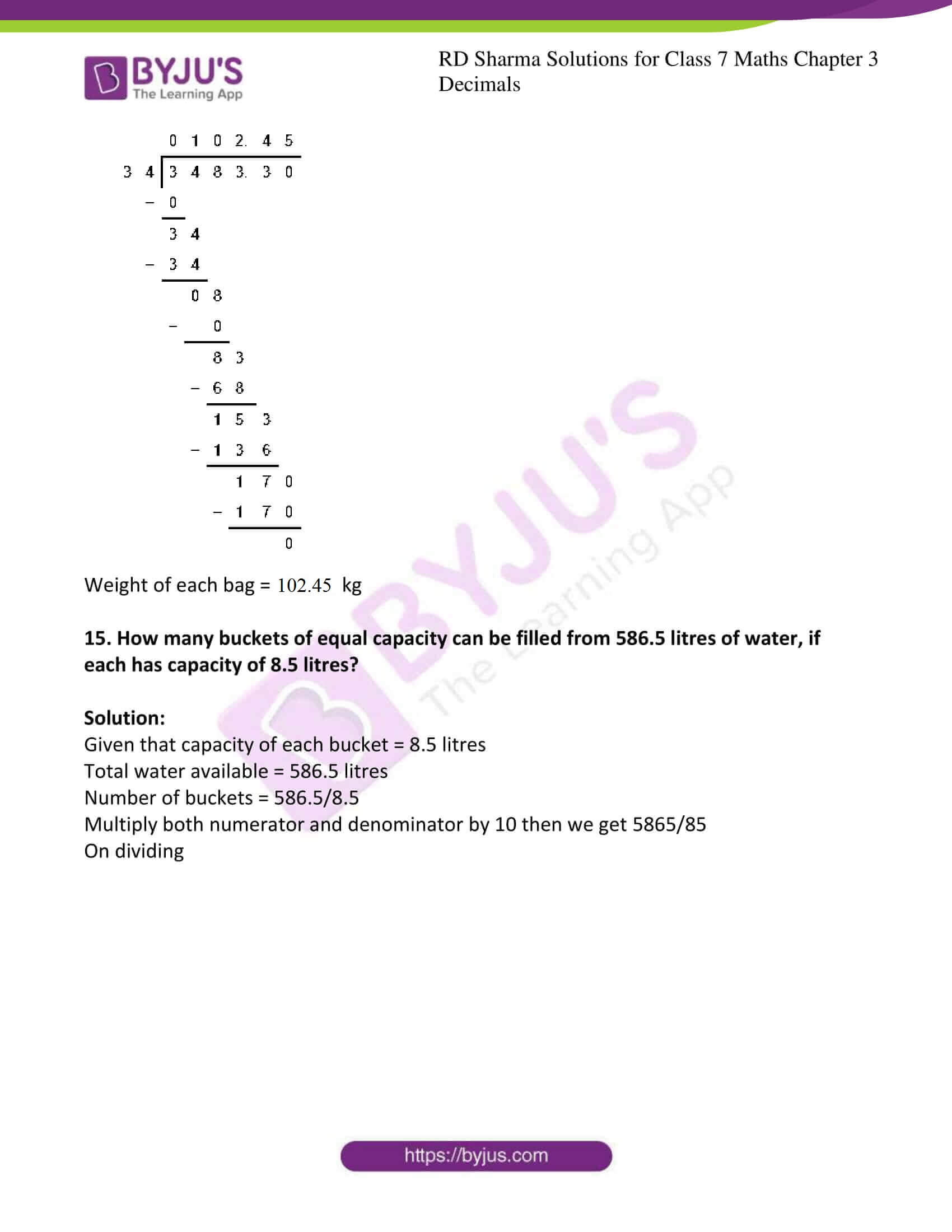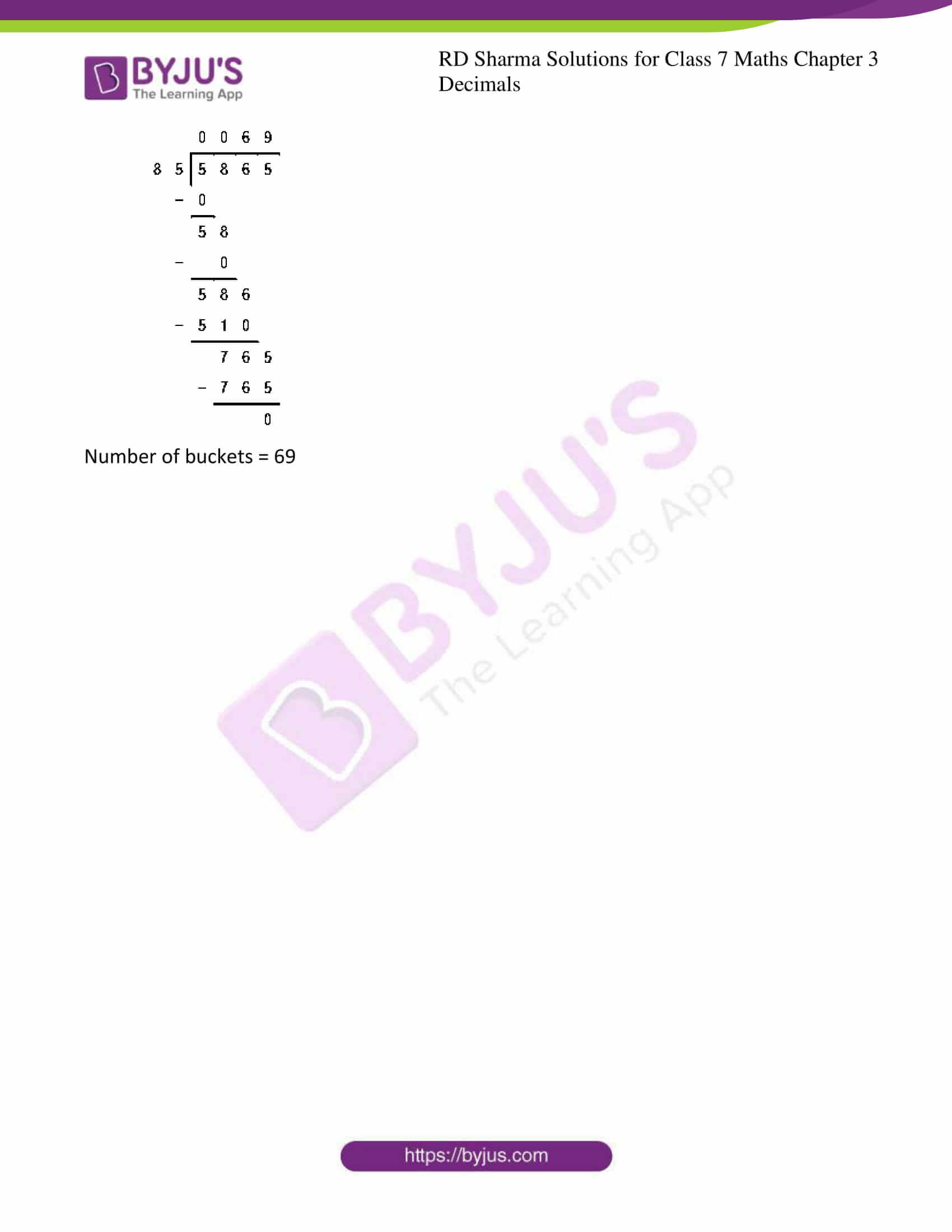## Exercise 3.1 Page No: 3.4

1. Write each of the following as decimals:

(i) (8/100)

(ii) 20 + (9/10) + (4/100)

(iii) 23 + (2/10) + (6/1000)

Solution:

(i) Given (8/100)

Mark the decimal point two places from right to left

(8/100) = 0.08

(ii) Given 20 + (9/10) + (4/100)

First convert the fractions (9/10) and (4/100) to decimals

Consider (9/10)

Mark the decimal point one place from right to left

(9/10) = 0.9

Now consider (4/100)

Mark the decimal point two places from right to left

(4/100) = 0.04

20 + (9/10) + (4/100) = 20 + 0.9 + 0.04

= 20.94

(iii) Given 23 + (2/10) + (6/1000)

First convert the fractions (2/10) and (6/1000) to decimals

Consider (2/10)

Mark the decimal point one place from right to left

(2/10) = 0.2

Now consider (6/1000)

Mark the decimal point three places from right to left

(6/1000) = 0.006

23 + (2/10) + (6/1000) = 23 + 0.2 + 0.006

= 23.206

2. Convert each of the following decimals as fractions:

(i) 0.04

(ii) 2.34

(iii) 0.342

(iv) 17.38

Solution:

(i) Given 0.04

Here we have to convert given decimals into fractions

0.04 can be written as (0.04/1)

Now multiply both numerator and denominator by 100 then we get

(0.04/1) = (0.04 × 100 /1 × 100)

= (4/100)

= (1/25)

(ii) Given 2.34

Here we have to convert given decimals into fractions

2.34 can be written as (2.34/1)

Now multiply both numerator and denominator by 100 then we get

(2.34/1) = (2.34 × 100 /1 × 100)

= (234/100)

= (117/50)

(iii) Given 0.342

Here we have to convert given decimals into fractions

0.342 can be written as (0.342/1)

Now multiply both numerator and denominator by 1000 then we get

(0.342/1) = (0.342 × 1000 /1 × 1000)

= (342/1000)

= (171/500)

(iv) Given 17.38

Here we have to convert given decimals into fractions

17.38 can be written as (17.38/1)

Now multiply both numerator and denominator by 100 then we get

(17.38/1) = (17.38 × 100 /1 × 100)

= (1738/100)

= (869/50)

3. Express the following fractions as decimals:

(i) (23/10)

(ii)

$$\begin{array}{l}25\frac{1}{8}\end{array}$$

(iii)

$$\begin{array}{l}39\frac{7}{35}\end{array}$$

(iv)

$$\begin{array}{l}15\frac{1}{25}\end{array}$$

Solution:

(i) Given (23/10)

Divide 23 by 10 we get

(23/10) = 2.3

(ii) Given

$$\begin{array}{l}25\frac{1}{8}\end{array}$$

$$\begin{array}{l}25\frac{1}{8}\end{array}$$
can be written as

$$\begin{array}{l}25\frac{1}{8}\end{array}$$
= 25 + (1/8)

Consider (1/8),

Now multiply both numerator and denominator by 125 to get 1000 as denominator

$$\begin{array}{l}25\frac{1}{8}\end{array}$$
= 25 + (1/8) = 25 + (1 × 125 /8 × 125)

= 25 + (125/1000)

= 25 + 0.125

= 25.125

(iii) Given

$$\begin{array}{l}39\frac{7}{35}\end{array}$$

First convert given mixed fraction

$$\begin{array}{l}39\frac{7}{35}\end{array}$$
into improper fraction

$$\begin{array}{l}39\frac{7}{35}\end{array}$$
= 1372/35

By dividing we get

$$\begin{array}{l}39\frac{7}{35}\end{array}$$
= 39.2

(iv) Given

$$\begin{array}{l}15\frac{1}{25}\end{array}$$

$$\begin{array}{l}15\frac{1}{25}\end{array}$$
can be written as

$$\begin{array}{l}15\frac{1}{25}\end{array}$$
= 15 + (1/25)

Consider (1/25),

Now multiply both numerator and denominator by 4 to get 100 as denominator

$$\begin{array}{l}15\frac{1}{25}\end{array}$$
= 15 + (1/25) = 15 + (1 × 4 /25 × 4)

= 15 + (4/100)

= 15 + 0.04

= 15.04

(i) 41.8, 39.24, 5.01 and 62.6

(ii) 18.03, 146.3, .829 and 5.324

Solution:

(i) Given 41.8, 39.24, 5.01 and 62.6

41.8

39.24

5.01

+ 62.6

148.65

(ii) Given 18.03, 146.3, 0.829 and 5.324

18.03

146.3

0.829

+ 5.324

170.483

5. Find the value of:

(i) 9.756 – 6.28

(ii) 48.1 – 0.37

(iii) 108.032 – 86.8

(iv) 100 – 26.32

Solution:

(i) Given 9.756 – 6.28

9.756

– 6.28

3.476

(ii) Given 48.1 – 0.37

48.1

– 0.37

47.73

(iii) Given 108.032 – 86.8

108.032

– 86.8

21.232

(iv) Given 100 – 26.32

100.00

– 26.32

73.68

6. Take out of 3.547 from 7.2

Solution:

Given 3.547 from 7.2

7.2

– 3.547

3.653

7. What is to be added to 36.85 to get 59.41?

Solution:

Given 36.85 and 59.41

Let the unknown number be x

x + 36.85 = 59.41

x = 59.41 – 36.85

x = 22.56

Hence 22.56 is to be added to 36.85 to get 59.41

8. What is to be subtracted from 17.1 to get 2.051?

Solution:

Let the unknown number be x

Given that x is to be subtracted from 17.1 to get 2.051

17.1 – x = 2.051

17.1 – 2.051 = x

x = 17.1 – 2.051

x = 15.049

9. By how much should 34.79 be increased to get 70.15?

Solution:

Let x be the unknown number

x + 34.79 = 70.15

x = 70.15 – 34.79

x = 35.36

35.36 should be increased to 34.79 to get 70.15

10. By how much should 59.71 be decreased to get 34.58?

Solution:

Let x be the unknown number

59.71 – x =34.58

59.71 – 34.58 = x

x = 59.71 – 34.58

x = 25.13

25.13 should be decreased by 59.71 to get 34.58

## Exercise 3.2 Page No: 3.8

1. Find the product:

(i) 4.74 × 10

(ii) 0.45 × 10

(iii) 0.0215 × 10

(iv) 0.0054 × 10

Solution:

(i) Given 4.74 × 10

Here we have to do normal multiplication with shifting the decimal point by one place to the right

Therefore 4.74 × 10 = 47.4

(ii) Given 0.45 × 10

Here we have to do normal multiplication with shifting the decimal point by one place to the right

Therefore 0.45 × 10 = 4.5

(iii) Given 0.0215 × 10

Here we have to do normal multiplication with shifting the decimal point by one place to the right

Therefore 0.0215 × 10 = 0.215

(iv) Given 0.0054 × 10

Here we have to do normal multiplication with shifting the decimal point by one place to the right

Therefore 0.0054 × 10 = 0.054

2. Find the product:

(i) 35.853 × 100

(ii) 42.5 × 100

(iii) 12.075 × 100

(iv) 100 × 0.005

Solution:

(i) Given 35.853 × 100

Here we have to do normal multiplication with shifting the decimal point by two places to the right

Therefore 35.853 × 100 = 3585.3

(ii) Given 42.5 × 100

Here we have to do normal multiplication with shifting the decimal point by two places to the right

Therefore 42.5 × 100 = 4250

(iii) Given 12.075 × 100

Here we have to do normal multiplication with shifting the decimal point by two places to the right

Therefore 12.075 × 100 = 1207.50

(iv) Given 100 × 0.005

Here we have to do normal multiplication with shifting the decimal point by two places to the right

Therefore 100 × 0.005 = 0.5

3. Find the product:

(i) 2.506 × 1000

(ii) 20.708 × 1000

(iii) 0.0529 × 1000

(iv) 1000 × 0.1

Solution:

(i) Given 2.506 × 1000

Here we have to do normal multiplication with shifting the decimal point by three places to the right

Therefore 2.506 × 1000 = 2506

(ii) Given 20.708 × 1000

Here we have to do normal multiplication with shifting the decimal point by three places to the right

Therefore 20.708 × 1000 = 20708

(iii) Given 0.0529 × 1000

Here we have to do normal multiplication with shifting the decimal point by three places to the right

Therefore 0.0529 × 1000 = 52.9

(iv) Given 1000 × 0.1

Here we have to do normal multiplication with shifting the decimal point by three places to the right

Therefore 1000 × 0.1 = 100

4. Find the product:

(i) 3.14 × 17

(ii) 0.745 × 12

(iii) 28.73 × 47

(iv) 0.0415 × 59

Solution:

(i) Given 3.14 × 17

First multiply as usual without looking at the decimal point

3.14 × 17 = 578

Now mark the decimal point in the product to have one place of decimal as there in the given decimal

3.14 × 17 = 57.8

(ii) Given 0.745 × 12

First multiply as usual without looking at the decimal point

0.745 × 12 = 894

Now mark the decimal point in the product to have three places of decimal as there in the given decimal

0.745 × 12 = 8.94

(iii) Given 28.73 × 47

First multiply as usual without looking at the decimal point

28.73 × 47 = 135031

Now mark the decimal point in the product to have two places of decimal as there in the given decimal

28.73 × 47 = 1350.31

(iv) Given 0.0415 × 59

First multiply as usual without looking at the decimal point

0.0415 × 59 = 24485

Now mark the decimal point in the product to have four places of decimal as there in the given decimal

0.0415 × 59 = 2.4485

5. Find:

(i) 1.07 × 0.02

(ii) 211.9 × 1.13

(iii) 10.05 × 1.05

(iv) 13.01 × 5.01

Solution:

(i) Given 1.07 × 0.02

First multiply as usual without looking at the decimal point

1.07 × 0.02 = 00214

Sum of the decimals is 4

Now mark the decimal point in the product to have four places of decimal as there in the given decimal

1.07 × 0.02 = 0.0214

(ii) Given 211.9 × 1.13

First multiply as usual without looking at the decimal point

211.9 × 1.13 = 239447

Sum of the decimals is 3

Now mark the decimal point in the product to have three places of decimal as there in the given decimal

211.9 × 1.13 = 239.447

(iii) Given 10.05 × 1.05

First multiply as usual without looking at the decimal point

10.05 × 1.05 = 105525

Sum of the decimals is 4

Now mark the decimal point in the product to have four places of decimal as there in the given decimal

10.05 × 1.05 = 10.5525

(iv) Given 13.01 × 5.01

First multiply as usual without looking at the decimal point

13.01 × 5.01 = 651801

Sum of the decimals is 4

Now mark the decimal point in the product to have four places of decimal as there in the given decimal

13.01 × 5.01 = 65.1801

6. Find the area of a rectangle whose length is 5.5m and breadth is 3.4m.

Solution:

Given length of rectangle = 5.5m

Breadth of rectangle = 3.4 m

Area of rectangle = length × breadth

= 5.5 × 3.4

= 18.7 m2

7. If the cost of a book is Rs 25.57, find the cost 0f 24 such books.

Solution:

Given cost of a book is Rs 25.57

Cost of 24 books = 25.57 × 24

= Rs 618.00

8. A car covers a distance of 14.75km in one liter of petrol. How much distance it will cover in 15.5 liters of petrol?

Solution:

Given that distance covered by car in 1 liter of petrol = 14.75 km

Distance covered by car in 15.5 liters of petrol = 14.75 × 15.5

= 228.625 km

9. One kg of rice costs Rs 42.65. What will be the cost of 18.25 kg of rice?

Solution:

Given cost of 1kg of rice = 42.65

Cost of 18.25kg of rice = 42.65 × 18.25

= Rs 778.3625

10. One meter of cloth costs Rs 152.50. What is the cost of 10.75 meters of cloth?

Solution:

Given that cost of 1m cloth = Rs 152.50

Cost of 10.75 m of cloth = 152.50 × 10.75

= Rs 1639.375

## Exercise 3.3 Page No: 3.16

1. Divide:

(i) 142.45 by 10

(ii) 54.25 by 10

(iii) 3.45 by 10

(iv) 0.57 by 10

(v) 0.0043 by 10

(vi) 0.004 by 10

Solution:

(i) Given 142.45 by 10

Now shifting the decimal point by one place to the left we can get the result

142.45/10 = 14.245

(ii) Given 54.25 by 10

Now shifting the decimal point by one place to the left we can get the result

54.25/10 = 5.425

(iii) Given 3.45 by 10

Now shifting the decimal point by one place to the left we can get the result

3.45/10 = 0.345

(iv) Given 0.57 by 10

Now shifting the decimal point by one place to the left we can get the result

0.57/10 = 0.057

(v) Given 0.0043 by 10

Now shifting the decimal point by one place to the left we can get the result

0.0043/10 = 0.0043

(vi) Given 0.004 by 10

Now shifting the decimal point by one place to the left we can get the result

0.004/10 = 0.0004

2. Divide:

(i) 459.5 by 100

(ii) 74.3 by 100

(iii) 5.8 by 100

(iv) 0.7 by 100

(v) 0.48 by 100

(vi) 0.03 by 100

Solution:

(i) Given 459.5 by 100

Now shifting the decimal point by two places to the left we can get the result

459.5/100 = 4.595

(ii) Given 74.3 by 100

Now shifting the decimal point by two places to the left we can get the result

74.3/100 = 0.743

(iii) Given 5.8 by 100

Now shifting the decimal point by two places to the left we can get the result

5.8/100 = 0.058

(iv) Given 0.7 by 100

Now shifting the decimal point by two places to the left we can get the result

0.7/100 = 0.007

(v) Given 0.48 by 100

Now shifting the decimal point by two places to the left we can get the result

0.48/100 = 0.0048

(vi) Given 0.03 by 100

Now shifting the decimal point by two places to the left we can get the result

0.03 / 100 = 0.0003

3. Divide:

(i) 235. 41 by 1000

(ii) 29.5 by 1000

(iii) 3.8 by 1000

(iv) 0.7 by 1000

Solution:

(i) Given 235. 41 by 1000

Now shifting the decimal point by three places to the left we can get the result

235. 41/1000 = 0.23541

(ii) Given 29.5 by 1000

Now shifting the decimal point by three places to the left we can get the result

29.5/1000 = 0.0295

(iii) Given 3.8 by 1000

Now shifting the decimal point by three places to the left we can get the result

3.8 /1000 = 0.0038

(iv) Given 0.7 by 1000

Now shifting the decimal point by three places to the left we can get the result

0.7 /1000 = 0.0007

4. Divide:

(i) 0.45 by 9

(ii) 217.44 by 18

(iii) 319.2 by 2.28

(iv) 40.32 by 9.6

(v) 0.765 by 0.9

(vi) 0.768 by 1.6

Solution:

(i) Given 0.45 by 9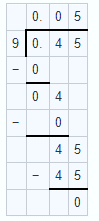0.45 by 9 = 0.05

(ii) Given 217.44 by 18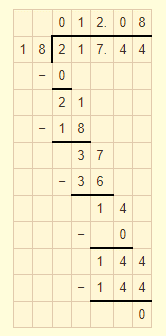217.44 by 18 = 12.08

3. Given 319.2 by 2.28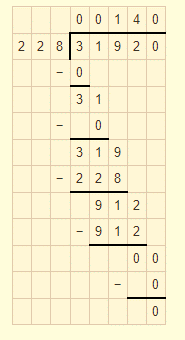319.2 by 2.28 = 140

(iv) Given 40.32 by 9.6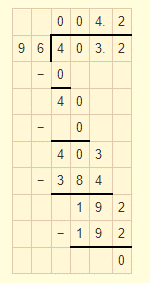40.32 by 9.6 = 4.2

(v) Given 0.765 by 0.9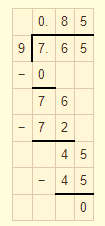0.765 by 0.9 = 0.85

(vi) Given 0.768 by 1.6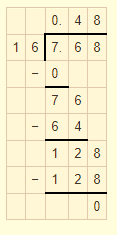0.768 by 1.6 = 0.48

5. Divide:

(i) 16.64 by 20

(ii) 0.192 by 12

(iii) 163.44 by 24

(iv) 403.2 by 96

(v) 16.344 by 12

(vi) 31.92 by 228

Solution:

(i) Given 16.64 by 20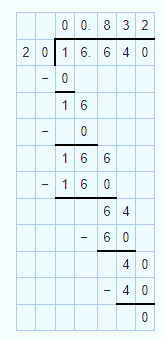16.64 by 20 = 0.832

(ii) Given 0.192 by 12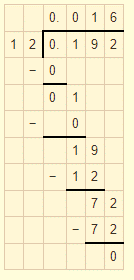0.192 by 12 = 0.016

(iii) Given 163.44 by 24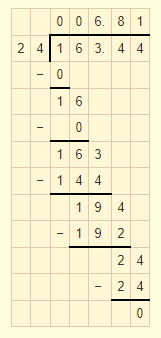163.44 by 24 = 6.81

(iv) Given 403.2 by 96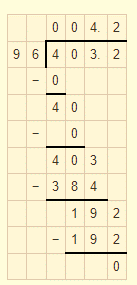403.2 by 96 = 4.2

(v) Given 16.344 by 12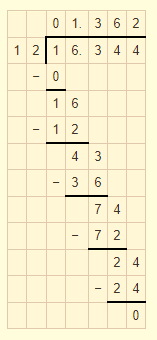16.344 by 12 = 1.362

(vi) Given 31.92 by 228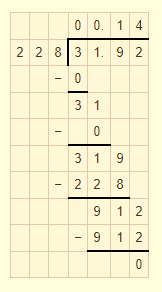31.92 by 228 = 0.14

6. Divide:

(i) 15.68 by 20

(ii) 164.6 by 200

(iii) 403.80 by 30

Solution:

(i) Given 15.68 by 20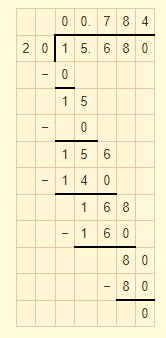15.68 by 20 = 0.784

(ii) Given 164.6 by 200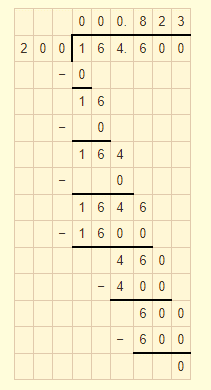164.6 by 200 = 0.823

(iii) Given 403.80 by 30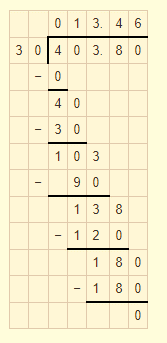403.80 by 30 = 13.46

7. Divide:

(i) 76 by 0.019

(ii) 88 by 0.08

(iii) 148 by 0.074

(iv) 7 by 0.014

Solution:

(i) Given 76 by 0.019

Multiply both numerator and denominator by 1000 then divide

= 76000/19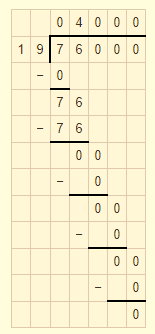76 by 0.019 = 4000

(ii) Given 88 by 0.08

Multiply both numerator and denominator by 100 then divide

= 8800/8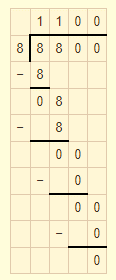88 by 0.08 = 1100

(iii) Given 148 by 0.074

Multiply both numerator and denominator by 1000 then divide

= 14800/74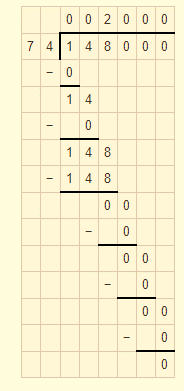148 by 0.074 = 2000

(iv) Given 7 by 0.014

Multiply both numerator and denominator by 1000 then divide

= 7000/14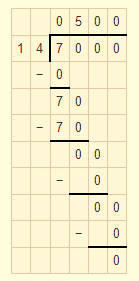7 by 0.014 = 500

8. Divide:

(i) 20 by 50

(ii) 8 by 100

(iii) 72 by 576

(iv) 144 by 15

Solution:

(i) Given 20 by 50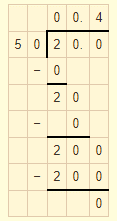20 by 50 = 0.4

(ii) Given 8 by 100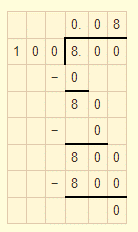8 by 100 = 0.08

(iii) Given 72 by 576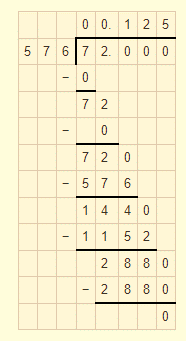72 by 576 = 0.125

(iv) Given 144 by 15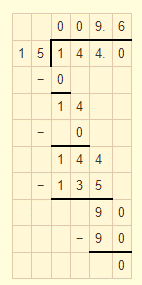144 by 15 = 9.6

9. A vehicle covers a distance 0f 43.2 km in 2.4 litres of petrol. How much distance will travel in 1 litre of petrol?

Solution:

Given distance covered by vehicle in 2.4 litres of petrol is = 43.2 km

Distance travel by vehicle in 1 litre of petrol = 43.2/2.4

Multiply both numerator and denominator by 10 then we get 432/24

On dividing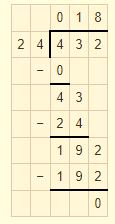Therefore 18km can travel in 1litre of petrol.

10. The total weight of some bags of wheat is 1743 kg. If each bag weighs 49.8 kg, how many bags are there?

Solution:

Given total weight of total bags = 1743 kg

Each bag weighs = 49.8 kg

Number of bags = 1743/49.8

Multiply both numerator and denominator by 10 then we get 17430/498

On dividing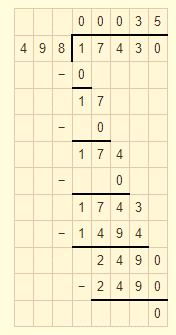Therefore number of bags are 35

11. Shikha cuts 50 m of cloth into pieces 0f 1.25 m each. How many pieces does she get?

Solution:

Given that total length of cloth = 50 m

Length of each piece = 1.25 m

Number of cloth piece = 50/1.25

Multiply both numerator and denominator by 100 then we get 5000/125

On dividing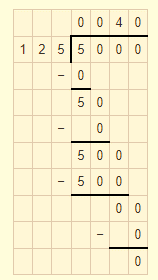Number of cloth piece = 40

12. Each side of a rectangular polygon is 2.5cm in length. The perimeter of the polygon is 12.5 cm. How many sides does the polygon have?

Solution:

Given that length of each side of polygon is = 2.5 cm

Perimeter of polygon = 12.5 cm

Number of sides = 12.5/2.5

Multiply both numerator and denominator by 10 then we get 125/25

On dividing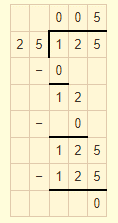Number of sides of polygon is 5

13. The product of two decimals is 42.987. If one of them is 12.46, find the other.

Solution:

Given that product of two decimals = 42.987

One of the number is = 12.46

Another number is = 42.987/12.46

Multiply both numerator and denominator by 1000 then we get 42987/12460

On dividing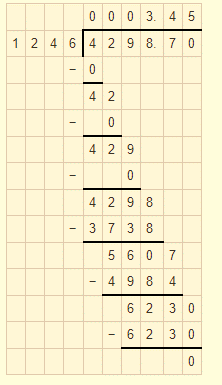Another number is 3.45

14. The weight of 34 bags of sugar is 3483.3 kg. If all bags weigh equally, find the weight of each bag.

Solution:

Given that weight of 34 bags of sugar is = 3483.3 kg

Weight of each bag = 3483.3/34

Multiply both numerator and denominator by 10 then we get 34833/34

On dividing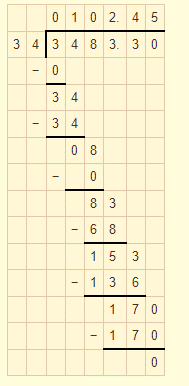Weight of each bag = 102.45 kg

15. How many buckets of equal capacity can be filled from 586.5 litres of water, if each has capacity of 8.5 litres?

Solution:

Given that capacity of each bucket = 8.5 litres

Total water available = 586.5 litres

Number of buckets = 586.5/8.5

Multiply both numerator and denominator by 10 then we get 5865/85

On dividing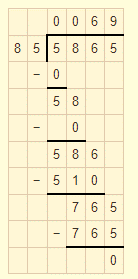Number of buckets = 69

## RD Sharma Solutions For Class 7 Maths Chapter 3 – Decimals

Chapter 3, Decimals contains three exercises. RD Sharma Solutions are given here which include the answers to all the questions present in these exercises. Let us have a look at some of the concepts that are being discussed in this chapter.

• Definitions of decimals
• Comparing decimals
• Types of decimals
• Addition and subtraction of decimals
• Multiplication of decimals
• Division of decimals

### Chapter brief of RD Sharma Solutions for Class 7 Maths Chapter 3 – Decimals

Chapter Decimals primarily discusses the fundamentals of decimals. Decimals can be considered as fractions whose denominators are 10, 100, 1000 etc. Here students will learn about the definition of decimals and their types, fundamental operations such as addition, subtraction, multiplication and division on them. This chapter clearly mentions different methods to solve these operations on decimals. Decimals are significant in our daily lives, as we purchase or sell something, it is compulsory to know about decimals. It is also an extended part of our number system.